Journal topic
Solid Earth, 10, 517–536, 2019
https://doi.org/10.5194/se-10-517-2019Special issue: Advances in seismic imaging across the scales

Solid Earth, 10, 517–536, 2019
https://doi.org/10.5194/se-10-517-2019Review article 11 Apr 2019

Review article | 11 Apr 2019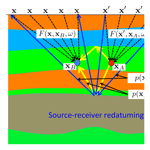# Green's theorem in seismic imaging across the scales

Green's theorem in seismic imaging across the scales
Kees Wapenaar, Joeri Brackenhoff, and Jan Thorbecke Kees Wapenaar et al.
• Department of Geoscience and Engineering, Delft University of Technology, Stevinweg 1, 2628 CN Delft, the Netherlands

Correspondence: Kees Wapenaar (c.p.a.wapenaar@tudelft.nl)

Abstract

The earthquake seismology and seismic exploration communities have developed a variety of seismic imaging methods for passive- and active-source data. Despite the seemingly different approaches and underlying principles, many of those methods are based in some way or another on Green's theorem. The aim of this paper is to discuss a variety of imaging methods in a systematic way, using a specific form of Green's theorem (the homogeneous Green's function representation) as a common starting point. The imaging methods we cover are time-reversal acoustics, seismic interferometry, back propagation, source–receiver redatuming and imaging by double focusing. We review classical approaches and discuss recent developments that fully account for multiple scattering, using the Marchenko method. We briefly indicate new applications for monitoring and forecasting of responses to induced seismic sources, which are discussed in detail in a companion paper.

1 Introduction

Through the years, the earthquake seismology and seismic exploration communities have developed a variety of seismic imaging methods for passive- and active-source data, based on a wide range of principles such as time-reversal acoustics, Green's function retrieval by noise correlation (a form of seismic interferometry), back propagation (also known as holography) and source–receiver redatuming. Many of these methods are rooted in some way or another in Green's theorem . The current paper is a modest attempt to discuss a variety of imaging methods and their underlying principles in a systematic way, using Green's theorem as the common starting point. We are certainly not the first to recognize links between different imaging methods. For example, discussed the link between back propagation and reverse-time migration, derived Green's function retrieval from the principle of time-reversal acoustics by physical reasoning and linked seismic interferometry to back propagation, to name but a few.

We start by reviewing a specific form of Green's theorem, namely the classical representation of the homogeneous Green's function, originally developed for optical holography . The homogeneous Green's function is the superposition of the causal Green's function and its time reversal. We use its surface-integral representation to derive time-reversal acoustics, seismic interferometry, back propagation, source–receiver redatuming and imaging by double focusing in a systematic way, confirming that these methods are all very similar. We briefly discuss the potential and the limitations of these methods. Because the classical homogeneous Green's function representation is based on a closed surface integral, an implicit assumption of all of these methods is that the medium of interest can be accessed from all sides. Due to the fact that acquisition is limited to the Earth's surface in most seismic applications, a major part of the closed surface integral is necessarily neglected. This implies that errors are introduced and, in particular, that multiple reflections between layer interfaces are not correctly handled. To address this issue, we also discuss a recently developed single-sided representation of the homogeneous Green's function. We use this to derive, in the same systematic way, modified seismic imaging methods that account for multiple reflections between layer interfaces. In a companion paper we extensively discuss applications for monitoring induced seismicity.

Although the solid Earth supports elastodynamic (vectorial) waves, to facilitate the comparison of the different methods discussed in this paper we have chosen to consider scalar waves only. Scalar waves, which obey the acoustic wave equation, serve as an approximation for compressional body waves propagating through the solid Earth, or for the fundamental mode of surface waves propagating along the Earth's surface, depending on the application. In several places we give references to extensions of the methods that account for the full elastodynamic wave field.

2 Theory and applications of a classical wave field representation

## 2.1 Classical homogeneous Green's function representation

We consider an inhomogeneous lossless acoustic medium, with mass density ρ(x) and compressibility κ(x), where $\mathbit{x}=\left({x}_{\mathrm{1}},{x}_{\mathrm{2}},{x}_{\mathrm{3}}\right)$ denotes the Cartesian coordinate vector. In this medium we define a unit impulsive point source of volume-injection rate density $q\left(\mathbit{x},t\right)=\mathit{\delta }\left(\mathbit{x}-{\mathbit{x}}_{\mathrm{A}}\right)\mathit{\delta }\left(t\right)$, where δ(⋅) denotes the Dirac delta function, xA represents the position of the source and t stands for time. The response to this source, observed at any position x in the inhomogeneous medium, is the Green's function $G\left(\mathbit{x},{\mathbit{x}}_{\mathrm{A}},t\right)$ and obeys the following wave equation:

$\begin{array}{}\text{(1)}& {\partial }_{i}\left({\mathit{\rho }}^{-\mathrm{1}}{\partial }_{i}G\right)-\mathit{\kappa }{\partial }_{t}^{\mathrm{2}}G=-\mathit{\delta }\left(\mathbit{x}-{\mathbit{x}}_{\mathrm{A}}\right){\partial }_{t}\mathit{\delta }\left(t\right),\end{array}$

where t stands for the temporal differential operator $\partial /\partial t$ and i represents the spatial differential operator $\partial /\partial {x}_{i}$. Latin subscripts (except t) take on the values 1, 2 and 3, and Einstein's summation convention applies to repeated subscripts. We impose the condition $G\left(\mathbit{x},{\mathbit{x}}_{\mathrm{A}},t\right)=\mathrm{0}$ for t<0, so that $G\left(\mathbit{x},{\mathbit{x}}_{\mathrm{A}},t\right)$ for t>0 is the causal solution of Eq. (1), representing a wave field originating from the source at xA. Note that the Green's function obeys source–receiver reciprocity, i.e., $G\left({\mathbit{x}}_{\mathrm{B}},{\mathbit{x}}_{\mathrm{A}},t\right)=G\left({\mathbit{x}}_{\mathrm{A}},{\mathbit{x}}_{\mathrm{B}},t\right)$, assuming both are causal and obey the same boundary conditions . This property will be frequently used without always mentioning it explicitly.

The time-reversal of the Green's function, $G\left(\mathbit{x},{\mathbit{x}}_{\mathrm{A}},-t\right)$, is the acausal solution of Eq. (1), which, for t<0, represents a wave field converging to a sink at xA. The homogeneous Green's function ${G}_{\mathrm{h}}\left(\mathbit{x},{\mathbit{x}}_{\mathrm{A}},t\right)$ is defined as the superposition of the Green's function and its time reversal, according to

$\begin{array}{}\text{(2)}& {G}_{\mathrm{h}}\left(\mathbit{x},{\mathbit{x}}_{\mathrm{A}},t\right)=G\left(\mathbit{x},{\mathbit{x}}_{\mathrm{A}},t\right)+G\left(\mathbit{x},{\mathbit{x}}_{\mathrm{A}},-t\right).\end{array}$

It is called “homogeneous” because it obeys a homogeneous wave equation, i.e., a wave equation without a singularity on the right-hand side. Hence ${\partial }_{i}\left({\mathit{\rho }}^{-\mathrm{1}}{\partial }_{i}{G}_{\mathrm{h}}\right)-\mathit{\kappa }{\partial }_{t}^{\mathrm{2}}{G}_{\mathrm{h}}=\mathrm{0}$, in which the medium parameters ρ(x) and κ(x) are generally not homogeneous. Note that in this paper we use the adjective “homogeneous” in two different ways. We define the Fourier transform of a time-dependent function u(t) as

$\begin{array}{}\text{(3)}& u\left(\mathit{\omega }\right)=\underset{-\mathrm{\infty }}{\overset{\mathrm{\infty }}{\int }}u\left(t\right)\mathrm{exp}\left(i\mathit{\omega }t\right)\mathrm{d}t.\end{array}$

Here ω denotes angular frequency and i the imaginary unit. For notational convenience, we use the same symbol for quantities in the time domain and in the frequency domain. The wave equation for the Green's function in the frequency domain reads

$\begin{array}{}\text{(4)}& {\partial }_{i}\left({\mathit{\rho }}^{-\mathrm{1}}{\partial }_{i}G\right)+\mathit{\kappa }{\mathit{\omega }}^{\mathrm{2}}G=i\mathit{\omega }\mathit{\delta }\left(\mathbit{x}-{\mathbit{x}}_{\mathrm{A}}\right).\end{array}$

The homogeneous Green's function in the frequency domain is defined as

$\begin{array}{ll}{G}_{\mathrm{h}}\left(\mathbit{x},{\mathbit{x}}_{\mathrm{A}},\mathit{\omega }\right)& =G\left(\mathbit{x},{\mathbit{x}}_{\mathrm{A}},\mathit{\omega }\right)+{G}^{*}\left(\mathbit{x},{\mathbit{x}}_{\mathrm{A}},\mathit{\omega }\right)\\ \text{(5)}& & =\mathrm{2}\mathrm{\Re }\mathit{\left\{}G\left(\mathbit{x},{\mathbit{x}}_{\mathrm{A}},\mathit{\omega }\right)\mathit{\right\}},\end{array}$

where the superscript asterisk denotes complex conjugation, and means that the real part is taken. The classical representation of the homogeneous Green's function reads (; ; Supplement, Sect. S1.3)

$\begin{array}{ll}{G}_{\mathrm{h}}\left({\mathbit{x}}_{\mathrm{B}},{\mathbit{x}}_{\mathrm{A}},\mathit{\omega }\right)& =\underset{\mathbb{S}}{\oint }\frac{\mathrm{1}}{i\mathit{\omega }\mathit{\rho }\left(\mathbit{x}\right)}\left(\mathit{\left\{}{\partial }_{i}G\left(\mathbit{x},{\mathbit{x}}_{\mathrm{B}},\mathit{\omega }\right)\mathit{\right\}}{G}^{*}\left(\mathbit{x},{\mathbit{x}}_{\mathrm{A}},\mathit{\omega }\right)\\ \text{(6)}& & -G\left(\mathbit{x},{\mathbit{x}}_{\mathrm{B}},\mathit{\omega }\right){\partial }_{i}{G}^{*}\left(\mathbit{x},{\mathbit{x}}_{\mathrm{A}},\mathit{\omega }\right)\right){n}_{i}\mathrm{d}\mathbit{x},\end{array}$

see Fig. 1. Here 𝕊 is an arbitrarily shaped closed surface with an outward pointing normal vector $\mathbit{n}=\left({n}_{\mathrm{1}},{n}_{\mathrm{2}},{n}_{\mathrm{3}}\right)$, which does not necessarily coincide with the boundary of the medium. It is assumed that xA and xB are situated inside 𝕊. Note that the aforementioned authors use a slightly different definition of the Green's function (the factor iω in the source term in Eq. (4) is absent in their case). Nevertheless, we will refer to Eq. (6) as the classical homogeneous Green's function representation. When 𝕊 is sufficiently smooth and the medium outside 𝕊 is homogeneous (with mass density ρ0, compressibility κ0 and propagation velocity ${c}_{\mathrm{0}}=\left({\mathit{\kappa }}_{\mathrm{0}}{\mathit{\rho }}_{\mathrm{0}}{\right)}^{-\mathrm{1}/\mathrm{2}}$), the two terms under the integral in Eq. (6) are nearly identical (but opposite in sign); hence, this representation may be approximated by

$\begin{array}{}\text{(7)}& {G}_{\mathrm{h}}\left({\mathbit{x}}_{\mathrm{B}},{\mathbit{x}}_{\mathrm{A}},\mathit{\omega }\right)=-\mathrm{2}\underset{\mathbb{S}}{\oint }\frac{\mathrm{1}}{i\mathit{\omega }{\mathit{\rho }}_{\mathrm{0}}}G\left(\mathbit{x},{\mathbit{x}}_{\mathrm{B}},\mathit{\omega }\right){\partial }_{i}{G}^{*}\left(\mathbit{x},{\mathbit{x}}_{\mathrm{A}},\mathit{\omega }\right){n}_{i}\mathrm{d}\mathbit{x}.\end{array}$

The main approximation is that evanescent waves are neglected .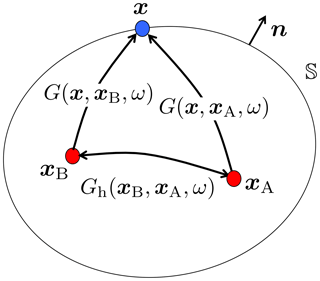Figure 1Configuration for the homogeneous Green's function representation (Eq. 6). The rays in this and subsequent figures represent the full responses between the source and receiver points, including primary and multiple scattering.

In the following sections we discuss different imaging methods. Each time we first introduce the specific method in an intuitive way, after which we present a more quantitative derivation based on Eq. (7).

## 2.2 Time-reversal acoustics

Time-reversal acoustics has been pioneered by Fink and co-workers . It makes use of the fact that the acoustic wave equation for a lossless medium is invariant under time reversal (for discussions regarding elastodynamic time-reversal methods we refer the reader to ; ; ). Hence, given a particular solution of the wave equation, its time-reversal obeys the same wave equation. Figure 2 illustrates the principle (following , and ). In Fig. 2a, an impulsive source at xA emits a wave field which, after propagation through a highly scattering medium, is recorded by receivers at x on the surface 𝕊0. In the practice of time-reversal acoustics, 𝕊0 is a finite open surface. We discuss the limitations of this later. The recordings at 𝕊0 are denoted as ${v}_{\mathrm{n}}\left(\mathbit{x},{\mathbit{x}}_{\mathrm{A}},t\right)$, where vn stands for the normal component of the particle velocity. Note that these recordings are very complex due to multiple scattering in the medium. In Fig. 2b, the time-reversals of these complex recordings, ${v}_{\mathrm{n}}\left(\mathbit{x},{\mathbit{x}}_{\mathrm{A}},-t\right)$, are emitted from the surface 𝕊0 into the medium. After propagating through the same scattering medium, the field should focus at xA, i.e., at the position of the original source. Figure 2c shows a snapshot of the field at t=0, which indeed contains a focus at xA. Figure 2d shows a horizontal cross-section of the amplitudes at t=0 at the depth level of the focus (the solid blue curve with the sharp peak). For comparison, the dotted red curve shows the amplitude cross-section of the focus that is obtained with a similar time-reversal experiment in absence of scatterers. As the solid blue curve has a sharper peak than the dotted red curve, we can conclude that multiple scattering contributes to the formation of the focus in Fig. 2c. The scattering medium effectively widens the aperture angle, which explains the better focus.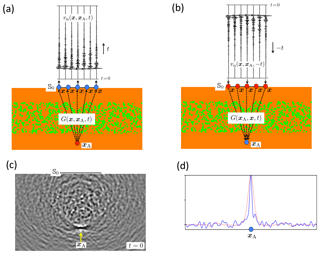Figure 2Principle of time-reversal acoustics. (a) Forward propagation from xA to the finite open surface 𝕊0. (b) Emission of the time-reversed recordings from 𝕊0 into the medium. (c) Snapshot of the wave field at t=0, with focus at xA. (d) Solid blue curve: amplitude cross-section of the focused field in (c), taken along a horizontal line through the focal point xA. Dotted red curve: amplitude cross-section obtained from a similar time-reversal experiment, in the absence of scatterers.

The time-reversal principle can be made more quantitative using Green's theorem (Fink2006). First, using the equation of motion, we express the normal component of the particle velocity at 𝕊 in the frequency domain as

$\begin{array}{}\text{(8)}& {v}_{\mathrm{n}}\left(\mathbit{x},{\mathbit{x}}_{\mathrm{A}},\mathit{\omega }\right)=\frac{\mathrm{1}}{i\mathit{\omega }{\mathit{\rho }}_{\mathrm{0}}}{\partial }_{i}G\left(\mathbit{x},{\mathbit{x}}_{\mathrm{A}},\mathit{\omega }\right){n}_{i}s\left(\mathit{\omega }\right),\end{array}$

where s(ω) is the spectrum of the source at xA. Using this in the homogeneous Green's function representation of Eq. (7) we obtain

$\begin{array}{}\text{(9)}& {G}_{\mathrm{h}}\left({\mathbit{x}}_{\mathrm{B}},{\mathbit{x}}_{\mathrm{A}},\mathit{\omega }\right){s}^{*}\left(\mathit{\omega }\right)=\mathrm{2}\underset{\mathbb{S}}{\oint }G\left({\mathbit{x}}_{\mathrm{B}},\mathbit{x},\mathit{\omega }\right){v}_{\mathrm{n}}^{*}\left(\mathbit{x},{\mathbit{x}}_{\mathrm{A}},\mathit{\omega }\right)\mathrm{d}\mathbit{x},\end{array}$

or, in the time domain (using Eq. 2),

$\begin{array}{ll}\mathit{\left\{}G\left({\mathbit{x}}_{\mathrm{B}},{\mathbit{x}}_{\mathrm{A}},t\right)& +G\left({\mathbit{x}}_{\mathrm{B}},{\mathbit{x}}_{\mathrm{A}},-t\right)\mathit{\right\}}*s\left(-t\right)\\ \text{(10)}& & =\mathrm{2}\underset{\mathbb{S}}{\oint }\underset{\text{“propagator”}}{\underbrace{G\left({\mathbit{x}}_{\mathrm{B}},\mathbit{x},t\right)}}*\underset{\text{“secondary\hspace{0.17em}sources”}}{\underbrace{{v}_{\mathrm{n}}\left(\mathbit{x},{\mathbit{x}}_{\mathrm{A}},-t\right)}}\mathrm{d}\mathbit{x},\end{array}$

where the inline asterisk (*) denotes temporal convolution. This is the fundamental expression for time-reversal acoustics. The integrand on the right-hand side formulates the propagation of the time-reversed field ${v}_{\mathrm{n}}\left(\mathbit{x},{\mathbit{x}}_{\mathrm{A}},-t\right)$ through the inhomogeneous medium by the Green's function $G\left({\mathbit{x}}_{\mathrm{B}},\mathbit{x},t\right)$ from the sources at x on the boundary 𝕊 to any receiver position xB inside the medium. The integral is taken along all source positions x on the closed boundary 𝕊. The right-hand side resembles Huygens' principle, which states that each point of an incident wave field acts as a secondary source, except that here the secondary sources on 𝕊 consist of time-reversed measurements instead of an incident wave field. The left-hand side quantifies the field at any point xB inside 𝕊, which consists within the negative time of a backward propagating field $G\left({\mathbit{x}}_{\mathrm{B}},{\mathbit{x}}_{\mathrm{A}},-t\right)*s\left(-t\right)$, converging to xA, and within the positive time of a forward propagating field $G\left({\mathbit{x}}_{\mathrm{B}},{\mathbit{x}}_{\mathrm{A}},t\right)*s\left(-t\right)$, originating from a virtual source at xA. By setting xB equal to xA we obtain the field at the focus (i.e., at the position of the original source). By taking xB variable in a small region around xA, while setting t equal to zero, Eq. (10) quantifies the focal spot. Assuming the source function s(t) is symmetric, this yields

$\begin{array}{}\text{(11)}& \left[\mathit{\left\{}G\left({\mathbit{x}}_{\mathrm{B}},{\mathbit{x}}_{\mathrm{A}},t\right)+G\left({\mathbit{x}}_{\mathrm{B}},{\mathbit{x}}_{\mathrm{A}},-t\right)\mathit{\right\}}*s\left(t\right){\right]}_{t=\mathrm{0}}=-\frac{\overline{\mathit{\rho }}}{\mathrm{2}\mathit{\pi }r}\stackrel{\mathrm{˙}}{s}\left(d/\overline{c}\right)\end{array}$

, where $\overline{c}$ and $\overline{\mathit{\rho }}$ are the propagation velocity and mass density in the neighborhood of xA, d is the distance of xB to xA, and $\stackrel{\mathrm{˙}}{s}\left(t\right)$ denotes the derivative of the source function s(t).

It should be noted that the integration in Eq. (10) takes place over sources on a closed surface 𝕊. However, in the example in Fig. 2 the time-reversed field is emitted into the medium from a finite open surface 𝕊0. Despite this discrepancy, a good focus is obtained around xA. Nevertheless, Fig. 2c also shows a noisy field at t=0, particularly in the scattering region. According to Eq. (10), this noisy field would vanish if the time-reversed field was emitted from a closed surface into the medium.

Figure 2 is representative of ultrasonic applications of time-reversal acoustics, because in those applications it is feasible to physically emit the time-reversed field into the real medium . Time-reversal acoustics also finds applications in geophysics at various scales, but in those applications the time-reversed field is emitted numerically into a model of the Earth. This is used for source characterization and for structural imaging by reverse-time migration . In these model-driven applications it is much more difficult to account for multiple scattering, which is therefore usually ignored. Moreover, the scattering mechanism is often very different, particularly in applications dedicated to imaging the Earth's crust. We discuss a second time-reversal example to illustrate this.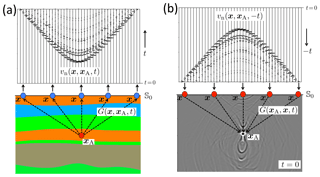Figure 3Time-reversal acoustics in a layered medium. (a) Forward propagation from xA to the finite open surface 𝕊0. (b) Emission of the time-reversed recordings from 𝕊0 into the medium and a snapshot of the wave field at t=0, with focus at xA.

Whereas in Fig. 2 we considered short-period multiple scattering at randomly distributed point-like scatterers in a homogeneous background medium, in Fig. 3 we consider long-period multiple scattering at extended interfaces between layers with distinct medium parameters (which is representative for multiple scattering in the Earth's crust). Figure 3a shows the response ${v}_{\mathrm{n}}\left(\mathbit{x},{\mathbit{x}}_{\mathrm{A}},t\right)$ to a source at xA inside a layered medium, observed at the surface 𝕊0. The time-reversal of this response is emitted from 𝕊0 into the same layered medium. The field at t=0 is shown in Fig. 3b. We again observe a clear focus at xA, but this time the multiple scattering does not contribute to the resolution of the focus (because there are no point scatterers that effectively widen the aperture angle). On the contrary, the multiply scattered waves give rise to strong, distinct artefacts in other regions in the medium. Again, these artefacts would disappear entirely if the time-reversed field was emitted from a closed surface, but this is of course unrealistic for geophysical applications. In Sect. 3.2 we discuss a modified approach to single-sided time-reversal acoustics which does not suffer from artefacts such as those in Fig. 3b.

## 2.3 Seismic interferometry

Under certain conditions, the cross-correlation of passive ambient-noise recordings at two receivers converges to the response that would be measured at one of the receivers if there were an impulsive source at the position of the other. This methodology, which creates a virtual source at the position of an actual receiver, is known as Green's function retrieval by noise correlation (a form of seismic interferometry). At the ultrasonic scale it has been pioneered by Weaver and co-workers , and the object of investigation at this scale is often a closed system (i.e., a finite specimen with reflecting boundaries on all sides). Early applications for open systems are discussed by Aki (1957), , , , , , , , , , , , and . A detailed discussion of the many aspects of seismic interferometry is beyond the scope of this paper. Overviews of seismic interferometry, for passive as well as controlled-source data, are given by , and .

Figure 4 illustrates the principle for passive ambient-noise data. In Fig. 4a, a distribution of uncorrelated noise sources N(x,t) at some finite open surface 𝕊0 emits waves through an inhomogeneous medium to receivers at xA and xB. The cross-correlation of the responses at xA and xB converges to $G\left({\mathbit{x}}_{\mathrm{B}},{\mathbit{x}}_{\mathrm{A}},t\right)*{C}_{N}\left(t\right)$, where CN(t) is the autocorrelation of the noise. The result is shown in Fig. 4b, for a fixed virtual source at xA and an array of receivers at variable xB.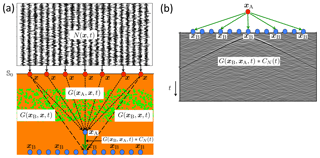Figure 4Principle of seismic interferometry. (a) Propagation of ambient noise from 𝕊0 through an inhomogeneous medium to receivers at xA and xB. (b) Cross-correlation of responses at xA and xB (with xA fixed and xB variable).

We use the homogeneous Green's function representation of Eq. (7) to explain this in a more quantitative way . Representations for elastodynamic interferometry are discussed by , and . Applying source–receiver reciprocity to the Green's functions under the integral in Eq. (7), we obtain

$\begin{array}{}\text{(12)}& {G}_{\mathrm{h}}\left({\mathbit{x}}_{\mathrm{B}},{\mathbit{x}}_{\mathrm{A}},\mathit{\omega }\right)=-\frac{\mathrm{2}}{i\mathit{\omega }{\mathit{\rho }}_{\mathrm{0}}}\underset{\mathbb{S}}{\oint }G\left({\mathbit{x}}_{\mathrm{B}},\mathbit{x},\mathit{\omega }\right){\partial }_{i}{G}^{*}\left({\mathbit{x}}_{\mathrm{A}},\mathbit{x},\mathit{\omega }\right){n}_{i}\mathrm{d}\mathbit{x}.\end{array}$

The integrand can be interpreted as the Fourier transform of the cross-correlation of responses to sources at x on closed surface 𝕊, observed by receivers at xA and xB. Note that 𝕊 is the surface containing the sources; it is not the boundary of the medium. $G\left({\mathbit{x}}_{\mathrm{B}},\mathbit{x},\mathit{\omega }\right)$ is the response to a monopole source at x, and ${\partial }_{i}G\left({\mathbit{x}}_{\mathrm{A}},\mathbit{x},\mathit{\omega }\right){n}_{i}$ is the response to a dipole source at the same position. In most situations there will only be one type of source present at x; therefore, we approximate the dipole sources by monopole sources, using the far-field approximation:

$\begin{array}{}\text{(13)}& {\partial }_{i}G\left({\mathbit{x}}_{\mathrm{A}},\mathbit{x},\mathit{\omega }\right){n}_{i}\to \frac{i\mathit{\omega }|\mathrm{cos}\left(\mathit{\alpha }\left(\mathbit{x}\right)\right)|}{{c}_{\mathrm{0}}}G\left({\mathbit{x}}_{\mathrm{A}},\mathbit{x},\mathit{\omega }\right).\end{array}$

Here α(x) is the angle between the normal to 𝕊 at x and the ray from the source at x to the receiver at xA. When the medium inside 𝕊 is inhomogeneous, there will be multiple rays between x and xA; hence, the angle α(x) is not unique. Moreover, for passive interferometry the positions of the sources are usually unknown. For simplicity we ignore the $|\mathrm{cos}\left(\mathit{\alpha }\left(\mathbit{x}\right)\right)|$ term in Eq. (13) and substitute the remaining expression into Eq. (12). This yields

$\begin{array}{}\text{(14)}& {G}_{\mathrm{h}}\left({\mathbit{x}}_{\mathrm{B}},{\mathbit{x}}_{\mathrm{A}},\mathit{\omega }\right)\approx \frac{\mathrm{2}}{{\mathit{\rho }}_{\mathrm{0}}{c}_{\mathrm{0}}}\underset{\mathbb{S}}{\oint }G\left({\mathbit{x}}_{\mathrm{B}},\mathbit{x},\mathit{\omega }\right){G}^{*}\left({\mathbit{x}}_{\mathrm{A}},\mathbit{x},\mathit{\omega }\right)\mathrm{d}\mathbit{x},\end{array}$

or, in the time domain (using Eq. 2),

$\begin{array}{ll}G\left({\mathbit{x}}_{\mathrm{B}},{\mathbit{x}}_{\mathrm{A}},t\right)& +G\left({\mathbit{x}}_{\mathrm{B}},{\mathbit{x}}_{\mathrm{A}},-t\right)\\ \text{(15)}& & \approx \frac{\mathrm{2}}{{\mathit{\rho }}_{\mathrm{0}}{c}_{\mathrm{0}}}\underset{\mathbb{S}}{\oint }G\left({\mathbit{x}}_{\mathrm{B}},\mathbit{x},t\right)*G\left({\mathbit{x}}_{\mathrm{A}},\mathbit{x},-t\right)\mathrm{d}\mathbit{x}\end{array}$

(the approximation sign refers to the far-field approximation, with the term $|\mathrm{cos}\left(\mathit{\alpha }\left(\mathbit{x}\right)\right)|$ ignored). This expression shows that the Green's function and its time-reversal between xA and xB can be approximately retrieved from the cross-correlation of responses to impulsive monopole sources at x on 𝕊, followed by an integration along 𝕊. This expression, and variants of it, are used in situations where responses to individual transient sources are available . Next, we modify this expression for simultaneous noise sources. For a distribution of noise sources N(x,t) on 𝕊 (like in Fig. 4a), we can write the following for the observed fields at xA and xB:

$\begin{array}{}\text{(16)}& & p\left({\mathbit{x}}_{\mathrm{A}},t\right)=\underset{\mathbb{S}}{\oint }G\left({\mathbit{x}}_{\mathrm{A}},\mathbit{x},t\right)*N\left(\mathbit{x},t\right)\mathrm{d}\mathbit{x},\text{(17)}& & p\left({\mathbit{x}}_{\mathrm{B}},t\right)=\underset{\mathbb{S}}{\oint }G\left({\mathbit{x}}_{\mathrm{B}},{\mathbit{x}}^{\prime },t\right)*N\left({\mathbit{x}}^{\prime },t\right)\mathrm{d}{\mathbit{x}}^{\prime }.\end{array}$

Assuming the noise sources are mutually uncorrelated, they obey

$\begin{array}{}\text{(18)}& 〈N\left({\mathbit{x}}^{\prime },t\right)*N\left(\mathbit{x},-t\right)〉={\mathit{\delta }}_{\mathbb{S}}\left(\mathbit{x}-{\mathbit{x}}^{\prime }\right){C}_{N}\left(t\right),\end{array}$

where CN(t) is the autocorrelation of the noise (which is assumed to be the same for all sources), 〈⋅〉 stands for time averaging and ${\mathit{\delta }}_{\mathbb{S}}\left(\mathbit{x}-{\mathbit{x}}^{\prime }\right)$ is a 2-D delta function defined in 𝕊. Cross-correlation of the observed noise fields in xA and xB gives

$\begin{array}{ll}〈p\left({\mathbit{x}}_{\mathrm{B}},t\right)*p\left({\mathbit{x}}_{\mathrm{A}},-t\right)〉& =〈\underset{\mathbb{S}}{\oint }\underset{\mathbb{S}}{\oint }G\left({\mathbit{x}}_{\mathrm{B}},{\mathbit{x}}^{\prime },t\right)*N\left({\mathbit{x}}^{\prime },t\right)\\ \text{(19)}& & *G\left({\mathbit{x}}_{\mathrm{A}},\mathbit{x},-t\right)*N\left(\mathbit{x},-t\right)\mathrm{d}{\mathbit{x}}^{\prime }\mathrm{d}\mathbit{x}〉.\end{array}$

Using Eq. (18) this becomes

$\begin{array}{ll}& 〈p\left({\mathbit{x}}_{\mathrm{B}},t\right)*p\left({\mathbit{x}}_{\mathrm{A}},-t\right)〉\\ \text{(20)}& & \phantom{\rule{0.25em}{0ex}}=\underset{\mathbb{S}}{\oint }G\left({\mathbit{x}}_{\mathrm{B}},\mathbit{x},t\right)*G\left({\mathbit{x}}_{\mathrm{A}},\mathbit{x},-t\right)*{C}_{N}\left(t\right)\mathrm{d}\mathbit{x}.\end{array}$

Note that the right-hand side resembles that of Eq. (15). Hence, if we convolve both sides of Eq. (15) with CN(t), we can replace its right-hand side with the left-hand side of Eq. (20), according to

$\begin{array}{ll}\mathit{\left\{}G\left({\mathbit{x}}_{\mathrm{B}},{\mathbit{x}}_{\mathrm{A}},t\right)& +G\left({\mathbit{x}}_{\mathrm{B}},{\mathbit{x}}_{\mathrm{A}},-t\right)\mathit{\right\}}*{C}_{N}\left(t\right)\\ \text{(21)}& & \approx \frac{\mathrm{2}}{{\mathit{\rho }}_{\mathrm{0}}{c}_{\mathrm{0}}}〈p\left({\mathbit{x}}_{\mathrm{B}},t\right)*p\left({\mathbit{x}}_{\mathrm{A}},-t\right)〉.\end{array}$

Equation (21) (and its extension for elastodynamic waves) is the fundamental expression of Green's function retrieval from ambient noise in an open system. The right-hand side represents the cross-correlation of the ambient-noise responses at two receivers at xA and xB. The left-hand side consists of a superposition of the virtual-source response $G\left({\mathbit{x}}_{\mathrm{B}},{\mathbit{x}}_{\mathrm{A}},t\right)*{C}_{N}\left(t\right)$ and its time-reversal $G\left({\mathbit{x}}_{\mathrm{B}},{\mathbit{x}}_{\mathrm{A}},-t\right)*{C}_{N}\left(t\right)$. Originally this methodology was based on intuitive arguments and was only used to retrieve the direct wave between the two receivers. As Eq. (21) is derived from a representation which holds for an inhomogeneous medium, it follows that the retrieved response is that of the inhomogeneous medium, hence, in principle it includes scattering (this will be illustrated below with a numerical example). The derivation that leads to Eq. (21) also reveals the approximations underlying the methodology of Green's function retrieval.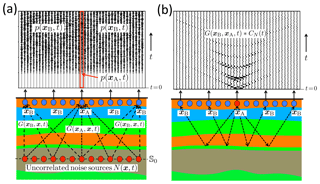Figure 5Seismic interferometry with body waves in a layered medium. The upper boundary is a free surface. (a) Noise observed by receivers just below the surface, due to uncorrelated noise sources in the subsurface (only the first 5 s of approximately 5 min of noise registrations are shown). (b) Retrieved reflection response, including multiple reflections.

According to Eqs. (16) and (17), it is assumed that the fields p(xA,t) and p(xB,t) are the responses to noise sources on a closed surface 𝕊. However, in the example in Fig. 4, the noise field is emitted into the medium from a finite open surface 𝕊0. A consequence of this discrepancy is that the retrieved response in Fig. 4b lacks the acausal term $G\left({\mathbit{x}}_{\mathrm{B}},{\mathbit{x}}_{\mathrm{A}},-t\right)*{C}_{N}\left(t\right)$. Moreover, the causal term $G\left({\mathbit{x}}_{\mathrm{B}},{\mathbit{x}}_{\mathrm{A}},t\right)*{C}_{N}\left(t\right)$ is blurred by scattering noise, which does not vanish with longer time-averaging. According to Eqs. (16), (17) and (21), the retrieved response would contain the causal and acausal terms and the scattering noise would vanish if the noise field was emitted from a closed surface and the recorded fields at xA and xB were correlated for a long enough time.

Figure 4 is representative of seismic surface-wave interferometry , in which case Fig. 4a should be interpreted as a plan view, with the noise signals representing microseisms, 𝕊0 representing a coast line and the Green's functions representing the fundamental mode of surface waves (with additional effort, higher-mode surface waves can be retrieved as well – ). The retrieved surface-wave Green's functions are typically used for tomographic imaging . Seismic interferometry can also be used for reflection imaging of the Earth's crust with body waves. Because the scattering mechanism is very different, we discuss a second example to illustrate seismic interferometry with body waves. Figure 5a shows the same layered medium as Fig. 3a, but this time with noise sources at 𝕊0 in the subsurface and with the upper surface being a free surface. For this situation the part of the closed-surface integral over the free surface in Eq. (6) vanishes. Hence, the closed surface integrals in Eqs. (16) and (17) can be replaced by open surface integrals over the noise sources in the subsurface in Fig. 5a. The responses to these noise sources, shown in the upper part of Fig. 5a, are recorded by receivers below the free surface. For p(xA,t) we take the central trace (indicated by the red box) and for p(xB,t) (with variable xB) all other traces. We apply Eq. (21) to obtain the virtual-source response $G\left({\mathbit{x}}_{\mathrm{B}},{\mathbit{x}}_{\mathrm{A}},t\right)*{C}_{N}\left(t\right)$ and its time-reversal $G\left({\mathbit{x}}_{\mathrm{B}},{\mathbit{x}}_{\mathrm{A}},-t\right)*{C}_{N}\left(t\right)$ for a fixed virtual source at xA and receivers at variable xB. The causal part is shown in Fig. 5b. In agreement with the theory, this is the full reflection response of the layered medium, including multiple reflections. Applications of reflection-response retrieval from ambient noise range from the shallow subsurface to the global scale . As body waves in ambient noise are usually weak in comparison with surface waves, much effort is spent on recovering the body waves from behind the surface waves. Reflection responses retrieved by body-wave interferometry are typically used for reflection imaging.

For both methods discussed here (surface-wave interferometry and body-wave interferometry) we assumed that the noise sources are regularly distributed along a part of 𝕊 and that they all have the same autocorrelation function. In many practical situations the source distribution is irregular, and the autocorrelations are different for different sources. Several approaches have been developed to account for these issues, such as iterative correlation , multidimensional deconvolution , directional balancing and generalized interferometry, circumventing Green's function retrieval .

## 2.4 Back propagation

Given a wave field observed at the surface of a medium, the field inside the medium can be obtained by back propagation . Because back propagation implies retrieving a 3-D field inside a volume from a 2-D field at a surface, it is also known as holography . Figure 6 illustrates the principle. In Fig. 6a, the field at the finite open surface 𝕊0 due to a source at xA inside a layered medium (the same medium as in Figs. 3 and 5) is back propagated to an arbitrary point xB inside the medium by the time-reversed direct arrival of the Green's function, ${G}_{\mathrm{d}}\left(\mathbit{x},{\mathbit{x}}_{\mathrm{B}},-t\right)$. Figure 6b shows ${G}_{\mathrm{d}}\left(\mathbit{x},{\mathbit{x}}_{\mathrm{B}},-t\right)$ (for fixed xB) and a snapshot of the back propagated field at time instant t1>0 for all xB. Note that above the source (which is located at xA) the primary upgoing field coming from the source is clearly retrieved. However, the field below the source is not retrieved. Moreover, several artefacts are present because multiple reflections between the layer interfaces are not accounted for.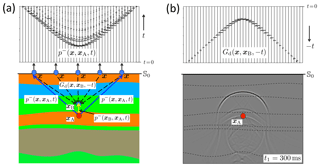Figure 6Principle of back propagation. (a) The upgoing wave field ${p}^{-}\left(\mathbit{x},{\mathbit{x}}_{\mathrm{A}},t\right)$ at the surface 𝕊0 and illustration of its back propagation to xB inside the medium. (b) The back propagation operator ${G}_{\mathrm{d}}\left(\mathbit{x},{\mathbit{x}}_{\mathrm{B}},-t\right)$ (for variable x along 𝕊0 and fixed xB) and a snapshot of the back propagated wave field ${p}^{-}\left({\mathbit{x}}_{\mathrm{B}},{\mathbit{x}}_{\mathrm{A}},t\right)$ at t1=300ms for all xB.

Back propagation is conceptually different from time-reversal acoustics. In time-reversal acoustics the observed wave field is reversed in time and (physically or numerically) emitted into the medium, whereas in back propagation the original observed wave field is numerically back-propagated through the medium by a time-reversed Green's function. Despite this conceptual difference (time reversal of the wave field versus time reversal of the propagation operator), it is not surprising that these methods are very similar from a mathematical point of view.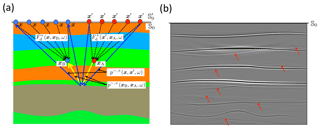Figure 7(a) Principle of source–receiver redatuming. (b) Reflectivity image $r\left({\mathbit{x}}_{\mathrm{A}}\right)\approx {p}^{-,+}\left({\mathbit{x}}_{\mathrm{A}},{\mathbit{x}}_{\mathrm{A}},t=\mathrm{0}\right)$ for all xA. The red arrows indicate erroneously imaged multiples.

A quantitative discussion of back propagation follows from Eq. (7). By interchanging xA and xB and multiplying both sides by the spectrum s(ω) of the source at xA, we obtain

$\begin{array}{ll}& {G}_{\mathrm{h}}\left({\mathbit{x}}_{\mathrm{B}},{\mathbit{x}}_{\mathrm{A}},\mathit{\omega }\right)s\left(\mathit{\omega }\right)\\ \text{(22)}& & \phantom{\rule{0.25em}{0ex}}=-\mathrm{2}\underset{\mathbb{S}}{\oint }\frac{\mathrm{1}}{i\mathit{\omega }{\mathit{\rho }}_{\mathrm{0}}}\mathit{\left\{}{\partial }_{i}{G}^{*}\left(\mathbit{x},{\mathbit{x}}_{\mathrm{B}},\mathit{\omega }\right)\mathit{\right\}}G\left(\mathbit{x},{\mathbit{x}}_{\mathrm{A}},\mathit{\omega }\right)s\left(\mathit{\omega }\right){n}_{i}\mathrm{d}\mathbit{x}.\end{array}$

Here $G\left(\mathbit{x},{\mathbit{x}}_{\mathrm{A}},\mathit{\omega }\right)s\left(\mathit{\omega }\right)$ stands for the observed field $p\left(\mathbit{x},{\mathbit{x}}_{\mathrm{A}},\mathit{\omega }\right)$ at the surface 𝕊, and $-\frac{\mathrm{2}}{i\mathit{\omega }{\mathit{\rho }}_{\mathrm{0}}}{\partial }_{i}{G}^{*}\left(\mathbit{x},{\mathbit{x}}_{\mathrm{B}},\mathit{\omega }\right){n}_{i}$ is the back propagation operator, both in the frequency domain. Hence, in theory the exact field ${G}_{\mathrm{h}}\left({\mathbit{x}}_{\mathrm{B}},{\mathbit{x}}_{\mathrm{A}},\mathit{\omega }\right)s\left(\mathit{\omega }\right)$ can be obtained at any xB inside the medium. Because in practical situations the field $p\left(\mathbit{x},{\mathbit{x}}_{\mathrm{A}},\mathit{\omega }\right)$ is only observed at a finite part 𝕊0 of the surface, approximations arise in practice when the closed surface 𝕊 is replaced by 𝕊0. One of the consequences is that multiple reflections are not handled correctly. A detailed analysis shows that the primary arrival of the upgoing wave field ${p}^{-}\left({\mathbit{x}}_{\mathrm{B}},{\mathbit{x}}_{\mathrm{A}},\mathit{\omega }\right)={G}^{-}\left({\mathbit{x}}_{\mathrm{B}},{\mathbit{x}}_{\mathrm{A}},\mathit{\omega }\right)s\left(\mathit{\omega }\right)$ is reasonably accurately retrieved with the following approximation of Eq. (22):

$\begin{array}{}\text{(23)}& {p}^{-}\left({\mathbit{x}}_{\mathrm{B}},{\mathbit{x}}_{\mathrm{A}},\mathit{\omega }\right)\approx \underset{{\mathbb{S}}_{\mathrm{0}}}{\int }{F}_{\mathrm{d}}^{+}\left(\mathbit{x},{\mathbit{x}}_{\mathrm{B}},\mathit{\omega }\right){p}^{-}\left(\mathbit{x},{\mathbit{x}}_{\mathrm{A}},\mathit{\omega }\right)\mathrm{d}\mathbit{x}.\end{array}$

Here the back propagation operator ${F}_{\mathrm{d}}^{+}\left(\mathbit{x},{\mathbit{x}}_{\mathrm{B}},\mathit{\omega }\right)$, also known as the focusing operator, is defined as

$\begin{array}{}\text{(24)}& {F}_{\mathrm{d}}^{+}\left(\mathbit{x},{\mathbit{x}}_{\mathrm{B}},\mathit{\omega }\right)=\frac{\mathrm{2}}{i\mathit{\omega }{\mathit{\rho }}_{\mathrm{0}}}{\partial }_{\mathrm{3}}{G}_{\mathrm{d}}^{*}\left(\mathbit{x},{\mathbit{x}}_{\mathrm{B}},\mathit{\omega }\right),\end{array}$

where we used ${n}_{\mathrm{3}}=-\mathrm{1}$ at 𝕊0, considering that the positive x3 axis is pointing downward. Equations (23) and (24) represent the common approach to back propagation for many applications in seismic imaging and inversion. It works well for primary waves in media with low contrasts, but it breaks down when the contrasts are strong and multiple reflections between the layer interfaces cannot be ignored. In Sect. 3.3 we discuss a modified approach to back-propagation which enables the recovery of the full wave field $p\left({\mathbit{x}}_{\mathrm{B}},{\mathbit{x}}_{\mathrm{A}},\mathit{\omega }\right)$, including the multiple reflections, inside the medium (also below the source at xA) and which also suppresses artefacts like those in Fig. 6b in a data-driven way.

## 2.5 Source–receiver redatuming and imaging by double focusing

In the previous section we discussed back propagation of ${p}^{-}\left(\mathbit{x},{\mathbit{x}}_{\mathrm{A}},\mathit{\omega }\right)$, which is the response to a source at xA inside the medium, observed at x at the surface. Here we extend this process for the situation in which both the sources and receivers are located at the surface. To this end, we first adapt Eqs. (23) and (24). We replace 𝕊0 with ${\mathbb{S}}_{\mathrm{0}}^{\prime }$ (just above 𝕊0), x with ${\mathbit{x}}^{\prime }\in {\mathbb{S}}_{\mathrm{0}}^{\prime }$, xA with x∈𝕊0 and xB with xA, and we add an extra superscript (+) to the wave fields (explained below), which yields

$\begin{array}{}\text{(25)}& {p}^{-,+}\left({\mathbit{x}}_{\mathrm{A}},\mathbit{x},\mathit{\omega }\right)\approx \underset{{\mathbb{S}}_{\mathrm{0}}^{\prime }}{\int }{F}_{\mathrm{d}}^{+}\left({\mathbit{x}}^{\prime },{\mathbit{x}}_{\mathrm{A}},\mathit{\omega }\right){p}^{-,+}\left({\mathbit{x}}^{\prime },\mathbit{x},\mathit{\omega }\right)\mathrm{d}{\mathbit{x}}^{\prime },\end{array}$

with

$\begin{array}{}\text{(26)}& {F}_{\mathrm{d}}^{+}\left({\mathbit{x}}^{\prime },{\mathbit{x}}_{\mathrm{A}},\mathit{\omega }\right)=\frac{\mathrm{2}}{i\mathit{\omega }{\mathit{\rho }}_{\mathrm{0}}}{\partial }_{\mathrm{3}}^{\prime }{G}_{\mathrm{d}}^{*}\left({\mathbit{x}}^{\prime },{\mathbit{x}}_{\mathrm{A}},\mathit{\omega }\right).\end{array}$

Here ${\partial }_{\mathrm{3}}^{\prime }$ stands for differentiation with respect to ${x}_{\mathrm{3}}^{\prime }$. In Eq. (25), ${p}^{-,+}\left({\mathbit{x}}^{\prime },\mathbit{x},\mathit{\omega }\right)={G}^{-,+}\left({\mathbit{x}}^{\prime },\mathbit{x},\mathit{\omega }\right)s\left(\mathit{\omega }\right)$ represents the reflection data at the surface. The first superscript () denotes that the field is upgoing at x; the second superscript (+) denotes that the source at x emits downgoing waves. Furthermore, ${p}^{-,+}\left({\mathbit{x}}_{\mathrm{A}},\mathbit{x},\mathit{\omega }\right)={G}^{-,+}\left({\mathbit{x}}_{\mathrm{A}},\mathbit{x},\mathit{\omega }\right)s\left(\mathit{\omega }\right)$ is the back propagated upgoing field at xA. Applying source–receiver reciprocity on both sides of Eq. (25) we obtain

$\begin{array}{}\text{(27)}& {p}^{-,+}\left(\mathbit{x},{\mathbit{x}}_{\mathrm{A}},\mathit{\omega }\right)\approx \underset{{\mathbb{S}}_{\mathrm{0}}^{\prime }}{\int }{p}^{-,+}\left(\mathbit{x},{\mathbit{x}}^{\prime },\mathit{\omega }\right){F}_{\mathrm{d}}^{+}\left({\mathbit{x}}^{\prime },{\mathbit{x}}_{\mathrm{A}},\mathit{\omega }\right)\mathrm{d}{\mathbit{x}}^{\prime }.\end{array}$

The receiver for upgoing waves at xA has turned into a source for downgoing waves at xA, and so on. Hence, Eq. (27) back propagates the sources from x on ${\mathbb{S}}_{\mathrm{0}}^{\prime }$ to xA. Substituting this into Eq. (23), with p replaced by ${p}^{-,+}$ on both sides, gives

$\begin{array}{ll}& {p}^{-,+}\left({\mathbit{x}}_{\mathrm{B}},{\mathbit{x}}_{\mathrm{A}},\mathit{\omega }\right)\\ \text{(28)}& & \phantom{\rule{0.25em}{0ex}}\approx \underset{{\mathbb{S}}_{\mathrm{0}}}{\int }\underset{{\mathbb{S}}_{\mathrm{0}}^{\prime }}{\int }{F}_{\mathrm{d}}^{+}\left(\mathbit{x},{\mathbit{x}}_{\mathrm{B}},\mathit{\omega }\right){p}^{-,+}\left(\mathbit{x},{\mathbit{x}}^{\prime },\mathit{\omega }\right){F}_{\mathrm{d}}^{+}\left({\mathbit{x}}^{\prime },{\mathbit{x}}_{\mathrm{A}},\mathit{\omega }\right)\mathrm{d}{\mathbit{x}}^{\prime }\mathrm{d}\mathbit{x}.\end{array}$

Here ${p}^{-,+}\left(\mathbit{x},{\mathbit{x}}^{\prime },\mathit{\omega }\right)$ represents the reflection response at the surface (illustrated by the blue arrows in Fig. 7a). Similarly, ${p}^{-,+}\left({\mathbit{x}}_{\mathrm{B}},{\mathbit{x}}_{\mathrm{A}},\mathit{\omega }\right)$ denotes the reflection response to a source for downgoing waves at xA, observed by a receiver for upgoing waves at xB (illustrated by the yellow arrows in Fig. 7a). According to Eq. (28), it is obtained by back propagating sources from x to xA with operator ${F}_{\mathrm{d}}^{+}\left({\mathbit{x}}^{\prime },{\mathbit{x}}_{\mathrm{A}},\mathit{\omega }\right)$ and receivers from x to xB with operator ${F}_{\mathrm{d}}^{+}\left(\mathbit{x},{\mathbit{x}}_{\mathrm{B}},\mathit{\omega }\right)$, indicated by the dashed arrows in Fig. 7a. In the exploration community this process is called (source–receiver) redatuming and is closely related to source–receiver interferometry . For the elastodynamic extension, see , and .

The redatumed response ${p}^{-,+}\left({\mathbit{x}}_{\mathrm{B}},{\mathbit{x}}_{\mathrm{A}},\mathit{\omega }\right)$ can be used for reflectivity imaging by setting xB equal to xA and selecting the t=0 component in the time domain, as follows:

$\begin{array}{}\text{(29)}& r\left({\mathbit{x}}_{\mathrm{A}}\right)\approx {p}^{-,+}\left({\mathbit{x}}_{\mathrm{A}},{\mathbit{x}}_{\mathrm{A}},t=\mathrm{0}\right)=\frac{\mathrm{1}}{\mathrm{2}\mathit{\pi }}\underset{-\mathrm{\infty }}{\overset{\mathrm{\infty }}{\int }}{p}^{-,+}\left({\mathbit{x}}_{\mathrm{A}},{\mathbit{x}}_{\mathrm{A}},\mathit{\omega }\right)\mathrm{d}\mathit{\omega }.\end{array}$

The combined process (Eqs. 28 and 29) comprises imaging by double focusing , because it involves the application of the focusing operator ${F}_{\mathrm{d}}^{+}\left(\mathbit{x},{\mathbit{x}}_{\mathrm{A}},\mathit{\omega }\right)$ twice. By taking the focal point xA variable, a reflectivity image of the entire region of interest is obtained. Figure 7b shows an image of the same layered medium as considered in previous examples obtained in this way. Note that the interfaces are clearly imaged, but also that significant artefacts are present because multiple reflections are not correctly handled (indicated by the red arrows). In Sect. 3.4 and 3.5 we discuss more rigorous approaches to source–receiver redatuming and imaging by double focusing, which account for multiple reflections in a data-driven way.

3 Theory and applications of a modified single-sided wave field representation

The applications of Green's theorem, discussed in Sect. 2, are all derived from the classical homogeneous Green's function representation. This representation is exact, but it involves an integral over a closed surface. In many practical situations the medium of interest is only accessible from one side, which implies that the integration can only be carried out over an open surface. This induces approximations, of which the incomplete treatment of multiple reflections is the most significant one. In the following we discuss a modification of the homogeneous Green's function representation which involves an integral over an open surface and yet accounts for all multiple reflections. We call this modified representation the single-sided homogeneous Green's function representation. Next, we discuss how it can be used to improve several of the applications discussed in Sect. 2.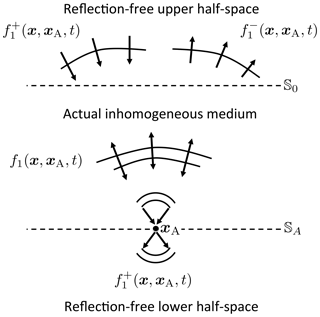Figure 8The focusing function ${f}_{\mathrm{1}}\left(\mathbit{x},{\mathbit{x}}_{\mathrm{A}},t\right)$ in a truncated version of the actual medium.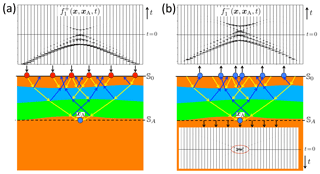Figure 9Numerical example of the focusing function. (a) Emission of the downgoing part of the focusing function from 𝕊0 into a truncated version of the actual medium. (b) Responses at 𝕊0 and 𝕊A.

## 3.1 Single-sided homogeneous Green's function representation

The classical homogeneous Green's function representations (Eqs. 6 and 7) are entirely formulated in terms of Green's functions and their time reversals. A Green's function is the causal response to a source at a specific position in space, say at xA. A time-reversed Green's function can be seen as a focusing function which focuses at xA. However, this only holds when it converges to xA equally from all directions, which can be achieved by emitting it into the medium from a closed surface. For practical situations we need another type of focusing function, which, when emitted into the medium from a single surface, focuses at xA. We introduce the focusing function using Fig. 8. This figure shows a truncated version of the medium, which is identical to the actual medium between the upper surface 𝕊0 and the focal plane 𝕊A (the plane which contains the focal point xA), but it is reflection free above 𝕊0 and below 𝕊A (here “reflection free” means that the medium parameters do not vary in the vertical direction). We call the focusing function ${f}_{\mathrm{1}}\left(\mathbit{x},{\mathbit{x}}_{\mathrm{A}},t\right)$. In the reflection-free half-space above 𝕊0 the focusing function consists of both a downgoing and upgoing part, according to

$\begin{array}{}\text{(30)}& {f}_{\mathrm{1}}\left(\mathbit{x},{\mathbit{x}}_{\mathrm{A}},t\right)={f}_{\mathrm{1}}^{+}\left(\mathbit{x},{\mathbit{x}}_{\mathrm{A}},t\right)+{f}_{\mathrm{1}}^{-}\left(\mathbit{x},{\mathbit{x}}_{\mathrm{A}},t\right),\end{array}$

where the superscripts + and indicate downgoing and upgoing, respectively. The downgoing part ${f}_{\mathrm{1}}^{+}\left(\mathbit{x},{\mathbit{x}}_{\mathrm{A}},t\right)$ is shaped such that ${f}_{\mathrm{1}}\left(\mathbit{x},{\mathbit{x}}_{\mathrm{A}},t\right)$ focuses at xA at t=0, and continues as a diverging downgoing field into the reflection-free half-space below 𝕊A. The upgoing part of the focusing function in the upper half-space, ${f}_{\mathrm{1}}^{-}\left(\mathbit{x},{\mathbit{x}}_{\mathrm{A}},t\right)$, is defined as the reflection response of the truncated medium to the downgoing focusing function ${f}_{\mathrm{1}}^{+}\left(\mathbit{x},{\mathbit{x}}_{\mathrm{A}},t\right)$. The focusing property at the focal plane 𝕊A can be formulated as

$\begin{array}{}\text{(31)}& \mathit{\delta }\left({\mathbit{x}}_{\mathrm{H},\mathrm{A}}^{\prime }-{\mathbit{x}}_{\mathrm{H},\mathrm{A}}\right)\mathit{\delta }\left(t\right)=\underset{{\mathbb{S}}_{\mathrm{0}}}{\int }\mathcal{T}\left({\mathbit{x}}_{\mathrm{A}}^{\prime },\mathbit{x},t\right)*{f}_{\mathrm{1}}^{+}\left(\mathbit{x},{\mathbit{x}}_{\mathrm{A}},t\right)\mathrm{d}\mathbit{x},\end{array}$

where $\mathcal{T}\left({\mathbit{x}}_{\mathrm{A}}^{\prime },\mathbit{x},t\right)$ is the transmission response of the truncated medium between 𝕊0 and 𝕊A, and xH,A and ${\mathbit{x}}_{\mathrm{H},\mathrm{A}}^{\prime }$ are the horizontal coordinates of xA and ${\mathbit{x}}_{\mathrm{A}}^{\prime }$ (both at 𝕊A), respectively (the precise definition of $\mathcal{T}\left({\mathbit{x}}_{\mathrm{A}}^{\prime },\mathbit{x},t\right)$ is given in Appendix A of ). In physical terms, Eq. (31) formulates the emission of ${f}_{\mathrm{1}}^{+}\left(\mathbit{x},{\mathbit{x}}_{\mathrm{A}},t\right)$ from 𝕊0 into the truncated medium, leading to a focus at xA. In mathematical terms, Eq. (31) defines ${f}_{\mathrm{1}}^{+}\left(\mathbit{x},{\mathbit{x}}_{\mathrm{A}},t\right)$ as the inverse of the transmission response $\mathcal{T}\left({\mathbit{x}}_{\mathrm{A}}^{\prime },\mathbit{x},t\right)$. Because the evanescent part of the transmission response cannot be inverted in a stable way, in practice the focusing function, and hence the focus at 𝕊A, is band-limited.

The focusing function is illustrated using a numerical example in Fig. 9. Figure 9a shows how the downgoing part of the focusing function, ${f}_{\mathrm{1}}^{+}\left(\mathbit{x},{\mathbit{x}}_{\mathrm{A}},t\right)$, is emitted from x at 𝕊0 into the medium. The first event (at negative time) propagates downward toward the focal point xA, indicated by the outer yellow rays (represented using arrows). On its path to the focal point it is reflected at layer interfaces, indicated by the blue rays. During upward propagation, these blue rays meet new yellow rays (coming from the later events of the focusing function), in such a way that effectively no downward reflection takes place at the layer interfaces, and so on. Hence, only the first event of the focusing function reaches the focal depth, where it focuses at xA. Figure 9b shows the responses to the focusing function, at 𝕊0 and 𝕊A. The response at 𝕊0 is the upgoing part of the focusing function, ${f}_{\mathrm{1}}^{-}\left(\mathbit{x},{\mathbit{x}}_{\mathrm{A}},t\right)$; the response at 𝕊A is the band-limited focused field.

Given the focusing function for a focal point at xA and the Green's function for a source at xB, the single-sided representation of the homogeneous Green's function in the frequency domain reads

$\begin{array}{ll}{G}_{\mathrm{h}}\left({\mathbit{x}}_{\mathrm{B}},{\mathbit{x}}_{\mathrm{A}},\mathit{\omega }\right)& =\mathrm{2}\underset{{\mathbb{S}}_{\mathrm{0}}}{\int }\frac{\mathrm{1}}{\mathit{\omega }\mathit{\rho }\left(\mathbit{x}\right)}\left(\mathit{\left\{}{\partial }_{i}{G}_{\mathrm{h}}\left(\mathbit{x},{\mathbit{x}}_{\mathrm{B}},\mathit{\omega }\right)\mathit{\right\}}\mathrm{\Im }\mathit{\left\{}{f}_{\mathrm{1}}\left(\mathbit{x},{\mathbit{x}}_{\mathrm{A}},\mathit{\omega }\right)\mathit{\right\}}\\ \text{(32)}& & -{G}_{\mathrm{h}}\left(\mathbit{x},{\mathbit{x}}_{\mathrm{B}},\mathit{\omega }\right)\mathrm{\Im }\mathit{\left\{}{\partial }_{i}{f}_{\mathrm{1}}\left(\mathbit{x},{\mathbit{x}}_{\mathrm{A}},\mathit{\omega }\right)\mathit{\right\}}\right){n}_{i}\mathrm{d}\mathbit{x},\end{array}$

where denotes the imaginary part. The derivation can be found in the Supplement, Sect. S2.2 (a similar single-sided representation for vectorial wave fields is derived by , and illustrated using numerical examples by ). In Eq. (32), 𝕊0 may be a curved surface. Moreover, the actual medium, in which the Green's function is defined, may be inhomogeneous above 𝕊0 (in addition to being inhomogeneous below 𝕊0). Note the resemblance with the classical representation of Eq. (6). Unlike the classical representation, which is exact, Eq. (32) holds under the assumption that evanescent waves can be neglected. When 𝕊0 is horizontal and the medium above 𝕊0 is homogeneous (for the Green's function as well as for the focusing function), this representation may be approximated by

$\begin{array}{ll}{G}_{\mathrm{h}}\left({\mathbit{x}}_{\mathrm{B}},{\mathbit{x}}_{\mathrm{A}},\mathit{\omega }\right)& =\mathrm{4}\mathrm{\Re }\underset{{\mathbb{S}}_{\mathrm{0}}}{\int }\frac{\mathrm{1}}{i\mathit{\omega }{\mathit{\rho }}_{\mathrm{0}}}G\left(\mathbit{x},{\mathbit{x}}_{\mathrm{B}},\mathit{\omega }\right){\partial }_{\mathrm{3}}\left({f}_{\mathrm{1}}^{+}\left(\mathbit{x},{\mathbit{x}}_{\mathrm{A}},\mathit{\omega }\right)\\ \text{(33)}& & -\mathit{\left\{}{f}_{\mathrm{1}}^{-}\left(\mathbit{x},{\mathbit{x}}_{\mathrm{A}},\mathit{\omega }\right){\mathit{\right\}}}^{*}\right)\mathrm{d}\mathbit{x}\end{array}$

. For the derivation, see the Supplement, Sect. S2.3. For the decomposed Green's function ${G}^{-,+}\left({\mathbit{x}}_{\mathrm{B}},{\mathbit{x}}_{\mathrm{A}},\mathit{\omega }\right)$, introduced in Sect. 2.5, we have the following representation (by combining Eqs. S31 and S38 of the Supplement)

$\begin{array}{ll}{G}^{-,+}& \left({\mathbit{x}}_{\mathrm{B}},{\mathbit{x}}_{\mathrm{A}},\mathit{\omega }\right)\\ & =\mathrm{2}\underset{{\mathbb{S}}_{\mathrm{0}}}{\int }\frac{\mathrm{1}}{i\mathit{\omega }{\mathit{\rho }}_{\mathrm{0}}}{G}^{-,+}\left(\mathbit{x},{\mathbit{x}}_{\mathrm{B}},\mathit{\omega }\right){\partial }_{\mathrm{3}}{f}_{\mathrm{1}}^{+}\left(\mathbit{x},{\mathbit{x}}_{\mathrm{A}},\mathit{\omega }\right)\mathrm{d}\mathbit{x}\\ \text{(34)}& & -\mathit{\chi }\left({\mathbit{x}}_{\mathrm{B}}\right){f}_{\mathrm{1}}^{-}\left({\mathbit{x}}_{\mathrm{B}},{\mathbit{x}}_{\mathrm{A}},\mathit{\omega }\right),\end{array}$

where χ is the characteristic function of the medium enclosed by 𝕊0 and 𝕊A. It is defined as

In many practical situations 𝕊0 is a free surface, which means that the assumption of a homogeneous medium above 𝕊0 is not fulfilled. A free surface gives rise to surface-related multiple reflections. These can be removed by a process called surface-related multiple elimination . Applying this process is equivalent to replacing the free surface with a transparent surface and a homogeneous half-space above this surface . Hence, when 𝕊0 is a free surface, Eqs. (33) and (34) hold for the situation after surface-related multiple elimination.

The representations of Eqs. (33) and (34) form the starting point for modifying several of the applications discussed in Sect. 2. These methods, which will be discussed in the subsequent sections, have the fact in common that they make use of focusing functions. As stated earlier, the focusing function ${f}_{\mathrm{1}}^{+}\left(\mathbit{x},{\mathbit{x}}_{\mathrm{A}},t\right)$ for x at 𝕊0 is the inverse of the transmission response of the truncated medium between 𝕊0 and 𝕊A. Hence, when a detailed model of the medium between these depth levels is available, its transmission response can be numerically modeled and ${f}_{\mathrm{1}}^{+}\left(\mathbit{x},{\mathbit{x}}_{\mathrm{A}},t\right)$ can be obtained by inverting this transmission response. Next, ${f}_{\mathrm{1}}^{-}\left(\mathbit{x},{\mathbit{x}}_{\mathrm{A}},t\right)$ can be obtained by applying the reflection response of the truncated medium to ${f}_{\mathrm{1}}^{+}\left(\mathbit{x},{\mathbit{x}}_{\mathrm{A}},t\right)$. This is obviously a model-driven approach. Conversely, when the reflection response of the actual medium is available at 𝕊0, the focusing functions ${f}_{\mathrm{1}}^{+}\left(\mathbit{x},{\mathbit{x}}_{\mathrm{A}},t\right)$ and ${f}_{\mathrm{1}}^{-}\left(\mathbit{x},{\mathbit{x}}_{\mathrm{A}},t\right)$ for x at 𝕊0 can be retrieved from this reflection response using a 3-D extension of the Marchenko method . This method needs an initial estimate of ${f}_{\mathrm{1}}^{+}\left(\mathbit{x},{\mathbit{x}}_{\mathrm{A}},t\right)$, for which one could use the inverse of the direct arrival of the transmission response. This requires only a smooth model of the medium between 𝕊0 and 𝕊A. In practice, the back propagating direct arrival of the Green's function, ${G}_{\mathrm{d}}\left(\mathbit{x},{\mathbit{x}}_{\mathrm{A}},-t\right)$, is usually taken as initial estimate. Because the Marchenko method uses the reflection response (obtained from reflection measurements at the surface 𝕊0) and a smooth model of the medium, it is a data-driven approach for retrieving the focusing functions. One of the underlying assumptions of the Marchenko method is that the Green's functions and the focusing functions are separable in time. This assumption is satisfied for layered media with moderate lateral variations (like in Fig. 3), considering moderate horizontal source–receiver offsets; it breaks down for strongly scattering media (like in Fig. 2). In the latter case the Marchenko method is only approximately valid, but despite the approximation it can still lead to better images than standard imaging methods . A further discussion of the 3-D Marchenko method is beyond the scope of this paper.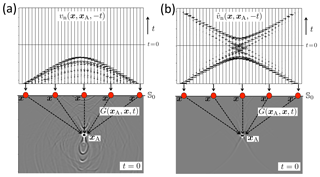Figure 10Time-reversal acoustics in a layered medium. (a) Classical approach: emission of the time-reversed recordings from 𝕊0 into the medium. (b) Emission of a modified field, defined by Eq. (36), into the medium. Note the improved focus.

## 3.2 Modified time-reversal acoustics

We discuss a modification of time-reversal acoustics. Assuming the focusing functions are available for x at 𝕊0 (for example, from the Marchenko method), we define a new particle velocity field, according to

$\begin{array}{ll}& {\stackrel{\mathrm{^}}{v}}_{\mathrm{n}}^{*}\left(\mathbit{x},{\mathbit{x}}_{\mathrm{A}},\mathit{\omega }\right)\\ \text{(36)}& & \phantom{\rule{0.25em}{0ex}}=\frac{\mathrm{1}}{i\mathit{\omega }{\mathit{\rho }}_{\mathrm{0}}}{\partial }_{\mathrm{3}}\left({f}_{\mathrm{1}}^{+}\left(\mathbit{x},{\mathbit{x}}_{\mathrm{A}},\mathit{\omega }\right)-\mathit{\left\{}{f}_{\mathrm{1}}^{-}\left(\mathbit{x},{\mathbit{x}}_{\mathrm{A}},\mathit{\omega }\right){\mathit{\right\}}}^{*}\right)s\left(\mathit{\omega }\right),\end{array}$

where for s(ω) we take a real-valued spectrum. Using this in Eq. (33) we obtain

$\begin{array}{}\text{(37)}& {G}_{\mathrm{h}}\left({\mathbit{x}}_{\mathrm{B}},{\mathbit{x}}_{\mathrm{A}},\mathit{\omega }\right)s\left(\mathit{\omega }\right)=\mathrm{4}\mathrm{\Re }\underset{{\mathbb{S}}_{\mathrm{0}}}{\int }G\left({\mathbit{x}}_{\mathrm{B}},\mathbit{x},\mathit{\omega }\right){\stackrel{\mathrm{^}}{v}}_{\mathrm{n}}^{*}\left(\mathbit{x},{\mathbit{x}}_{\mathrm{A}},\mathit{\omega }\right)\mathrm{d}\mathbit{x}.\end{array}$

In the time domain this becomes

$\begin{array}{ll}{G}_{\mathrm{h}}\left({\mathbit{x}}_{\mathrm{B}},{\mathbit{x}}_{\mathrm{A}},t\right)*s\left(t\right)& =\mathrm{2}\underset{{\mathbb{S}}_{\mathrm{0}}}{\int }G\left({\mathbit{x}}_{\mathrm{B}},\mathbit{x},t\right)*{\stackrel{\mathrm{^}}{v}}_{\mathrm{n}}\left(\mathbit{x},{\mathbit{x}}_{\mathrm{A}},-t\right)\mathrm{d}\mathbit{x}\\ \text{(38)}& & +\mathrm{2}\underset{{\mathbb{S}}_{\mathrm{0}}}{\int }G\left({\mathbit{x}}_{\mathrm{B}},\mathbit{x},-t\right)*{\stackrel{\mathrm{^}}{v}}_{\mathrm{n}}\left(\mathbit{x},{\mathbit{x}}_{\mathrm{A}},t\right)\mathrm{d}\mathbit{x}.\end{array}$

The first integral is the same as that in Eq. (10) (except that ${\stackrel{\mathrm{^}}{v}}_{\mathrm{n}}$ is defined differently), whereas the second integral is the time reversal of the first one. For ultrasonic applications, assuming there are receivers at one or more xB locations, the field ${\stackrel{\mathrm{^}}{v}}_{\mathrm{n}}\left(\mathbit{x},{\mathbit{x}}_{\mathrm{A}},-t\right)$ can be emitted physically into the real medium and its response can be measured at xB. The homogeneous Green's function is then obtained by superposing this response and its time reversal. For geophysical applications, the first integral can, at least in theory, be evaluated by numerically emitting the field ${\stackrel{\mathrm{^}}{v}}_{\mathrm{n}}\left(\mathbit{x},{\mathbit{x}}_{\mathrm{A}},-t\right)$ into a model of the Earth. The superposition of this integral and its time-reversal gives the homogeneous Green's function. Following either one of these procedures, the result obtained at t=0 is shown in Fig. 10b. For comparison, Fig. 10a once more shows the classical time-reversal result of Fig. 3b. Note the different character of the fields vn and ${\stackrel{\mathrm{^}}{v}}_{\mathrm{n}}$ in the upper panels, which only have one event in common i.e., the time-reversed direct arrival. The snapshots at t=0 in the lower panels are also very different: the artefacts in Fig. 10a are almost entirely absent in Fig. 10b. The latter figure only shows a clear focus at xA.

Obtaining an accurate focus as in Fig. 10b by numerically emitting the field ${\stackrel{\mathrm{^}}{v}}_{\mathrm{n}}\left(\mathbit{x},{\mathbit{x}}_{\mathrm{A}},-t\right)$ into the Earth requires a very accurate model of the Earth, which should include accurate information on the position, structure and contrast of the layer interfaces. This requirement can be overcome by also retrieving the Green's function $G\left({\mathbit{x}}_{\mathrm{B}},\mathbit{x},t\right)$ in Eq. (38) using the Marchenko method and evaluating the integrals for all xB. This is not discussed any further here. Alternative methods that do not require information about the layer interfaces are discussed in Sect. 3.3 to 3.5 and are illustrated using examples.

## 3.3 Modified back propagation

We modify the approach for back propagation. By interchanging xA and xB in Eq. (33) and multiplying both sides with a real-valued source spectrum s(ω), we obtain

$\begin{array}{ll}p\left({\mathbit{x}}_{\mathrm{B}},{\mathbit{x}}_{\mathrm{A}},\mathit{\omega }\right)& +{p}^{*}\left({\mathbit{x}}_{\mathrm{B}},{\mathbit{x}}_{\mathrm{A}},\mathit{\omega }\right)\\ \text{(39)}& & =\mathrm{2}\mathrm{\Re }\underset{{\mathbb{S}}_{\mathrm{0}}}{\int }F\left(\mathbit{x},{\mathbit{x}}_{\mathrm{B}},\mathit{\omega }\right)p\left(\mathbit{x},{\mathbit{x}}_{\mathrm{A}},\mathit{\omega }\right)\mathrm{d}\mathbit{x},\end{array}$

with $p\left(\mathbit{x},{\mathbit{x}}_{\mathrm{A}},\mathit{\omega }\right)=G\left(\mathbit{x},{\mathbit{x}}_{\mathrm{A}},\mathit{\omega }\right)s\left(\mathit{\omega }\right)$ and

$\begin{array}{}\text{(40)}& F\left(\mathbit{x},{\mathbit{x}}_{\mathrm{B}},\mathit{\omega }\right)=\frac{\mathrm{2}}{i\mathit{\omega }{\mathit{\rho }}_{\mathrm{0}}}{\partial }_{\mathrm{3}}\left({f}_{\mathrm{1}}^{+}\left(\mathbit{x},{\mathbit{x}}_{\mathrm{B}},\mathit{\omega }\right)-\mathit{\left\{}{f}_{\mathrm{1}}^{-}\left(\mathbit{x},{\mathbit{x}}_{\mathrm{B}},\mathit{\omega }\right){\mathit{\right\}}}^{*}\right).\end{array}$

Note that the operator ${F}_{\mathrm{d}}^{+}\left(\mathbit{x},{\mathbit{x}}_{\mathrm{B}},\mathit{\omega }\right)$ in Eq. (24) is an approximation of the operator $F\left(\mathbit{x},{\mathbit{x}}_{\mathrm{B}},\mathit{\omega }\right)$ in Eq. (40). It is obtained by omitting the term $\mathit{\left\{}{f}_{\mathrm{1}}^{-}\left(\mathbit{x},{\mathbit{x}}_{\mathrm{B}},\mathit{\omega }\right){\mathit{\right\}}}^{*}$ and replacing the term ${f}_{\mathrm{1}}^{+}\left(\mathbit{x},{\mathbit{x}}_{\mathrm{B}},\mathit{\omega }\right)$ by its initial estimate, i.e., the Fourier transform of the direct arrival of the Green's function, ${G}_{\mathrm{d}}\left(\mathbit{x},{\mathbit{x}}_{\mathrm{B}},-t\right)$. Figure 11 illustrates, in the time domain, the principle of modified back propagation. In Fig. 11a, the field $p\left(\mathbit{x},{\mathbit{x}}_{\mathrm{A}},t\right)$ is back propagated to an arbitrary point xB inside the medium by operator $F\left(\mathbit{x},{\mathbit{x}}_{\mathrm{B}},t\right)$. This operator can be obtained from reflection data at the surface and the initial estimate ${G}_{\mathrm{d}}\left(\mathbit{x},{\mathbit{x}}_{\mathrm{B}},-t\right)$, using the Marchenko method. Figure 11b shows $F\left(\mathbit{x},{\mathbit{x}}_{\mathrm{B}},t\right)$ (for fixed xB) and a snapshot of the back propagated field at a time instant t1>0 for all xB. Note that the full field $p\left({\mathbit{x}}_{\mathrm{B}},{\mathbit{x}}_{\mathrm{A}},t\right)$ is retrieved (downgoing and upgoing components, primaries and multiples) and that hardly any artefacts are visible. The dashed lines in the snapshot in Fig. 11b indicate the interfaces to aid with the interpretation of the snapshot. Note, however, that these interfaces need not be known to obtain this result: only a smooth subsurface model is required to define the initial estimate ${G}_{\mathrm{d}}\left(\mathbit{x},{\mathbit{x}}_{\mathrm{B}},-t\right)$ of the focusing operator. All other events in the focusing operator come directly from the reflection data at the surface.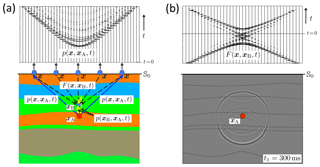Figure 11Principle of modified back propagation. (a) The wave field $p\left(\mathbit{x},{\mathbit{x}}_{\mathrm{A}},t\right)$ at the surface 𝕊0 and illustration of its back propagation to xB inside the medium. (b) The back propagation operator $F\left(\mathbit{x},{\mathbit{x}}_{\mathrm{B}},t\right)$ (for variable x along 𝕊0 and fixed xB) and a snapshot of the back propagated wave field $p\left({\mathbit{x}}_{\mathrm{B}},{\mathbit{x}}_{\mathrm{A}},t\right)$ at t1=300 ms for all xB.

This back propagation method has an interesting application in the monitoring of induced seismicity. Assuming $p\left(\mathbit{x},{\mathbit{x}}_{\mathrm{A}},t\right)$ stands for the response to an induced seismic source at xA, this method creates, in a data-driven way, omnidirectional virtual receivers at any xB to monitor the emitted field from the source to the surface. This application is extensively discussed in the companion paper .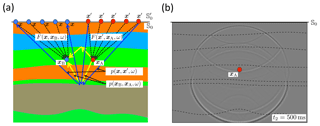Figure 12(a) Principle of modified source–receiver redatuming. (b) Snapshot of the wave field $p\left({\mathbit{x}}_{\mathrm{B}},{\mathbit{x}}_{\mathrm{A}},t\right)$ at t2=500ms for all xB.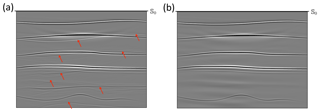Figure 13Reflectivity images obtained using the double-focusing method. (a) Classical approach. (b) Modified approach.

We modify the approach for source–receiver redatuming. First, in Eq. (39), we replace 𝕊0 with ${\mathbb{S}}_{\mathrm{0}}^{\prime }$ (just above 𝕊0), x with ${\mathbit{x}}^{\prime }\in {\mathbb{S}}_{\mathrm{0}}^{\prime }$, xA with x∈𝕊0 and xB with xA. Next, we apply source–receiver reciprocity on both sides of the equation. This yields

$\begin{array}{ll}p\left(\mathbit{x},{\mathbit{x}}_{\mathrm{A}},\mathit{\omega }\right)& +{p}^{*}\left(\mathbit{x},{\mathbit{x}}_{\mathrm{A}},\mathit{\omega }\right)\\ \text{(41)}& & =\mathrm{2}\mathrm{\Re }\underset{{\mathbb{S}}_{\mathrm{0}}^{\prime }}{\int }p\left(\mathbit{x},{\mathbit{x}}^{\prime },\mathit{\omega }\right)F\left({\mathbit{x}}^{\prime },{\mathbit{x}}_{\mathrm{A}},\mathit{\omega }\right)\mathrm{d}{\mathbit{x}}^{\prime };\end{array}$

$F\left({\mathbit{x}}^{\prime },{\mathbit{x}}_{\mathrm{A}},\mathit{\omega }\right)$ is defined as in Eq. (40), with 3 replaced by ${\partial }_{\mathrm{3}}^{\prime }$, similar to Eq. (26). The field $p\left(\mathbit{x},{\mathbit{x}}^{\prime },\mathit{\omega }\right)=G\left(\mathbit{x},{\mathbit{x}}^{\prime },\mathit{\omega }\right)s\left(\mathit{\omega }\right)$ represents the data at the surface. Equation (41) back propagates the sources from x on ${\mathbb{S}}_{\mathrm{0}}^{\prime }$ to xA. Source–receiver redatuming is now defined as the following two-step process. In step one, apply Eq. (41) to create an omnidirectional virtual source at any desired position xA in the subsurface. According to the left-hand side, the response to this virtual source is observed by actual receivers at x at the surface. Isolate $p\left(\mathbit{x},{\mathbit{x}}_{\mathrm{A}},\mathit{\omega }\right)$ from the left-hand side by applying a time window (a simple Heaviside function) in the time domain. In step two, substitute the retrieved response $p\left(\mathbit{x},{\mathbit{x}}_{\mathrm{A}},\mathit{\omega }\right)$ into Eq. (39) to create virtual receivers at any position xB in the subsurface. Figure 12a illustrates the principle. The operators can be obtained using the Marchenko method. Figure 12b shows a snapshot of $p\left({\mathbit{x}}_{\mathrm{B}},{\mathbit{x}}_{\mathrm{A}},t\right)$ at a time instant ${t}_{\mathrm{2}}>{t}_{\mathrm{1}}>\mathrm{0}$ for all xB (the retrieved snapshot at t1 is indistinguishable from that in Fig. 11b, which is why we chose to show a snapshot at another time instant). The dashed lines in the snapshot in Fig. 12b indicate the interfaces to aid with the interpretation of the snapshot, but the interfaces need not to be known to obtain this result. This method has an interesting application in forecasting the effects of induced seismicity. Assuming xA is the position where induced seismicity is likely to take place, this method forecasts the response by creating, in a data-driven way, a virtual source at xA and virtual receivers at any xB that observe the propagation and scattering of its emitted field from the source to the surface. This method is extensively discussed in the companion paper .

## 3.5 Modified imaging by double focusing

If we applied imaging to the retrieved response $p\left({\mathbit{x}}_{\mathrm{B}},{\mathbit{x}}_{\mathrm{A}},\mathit{\omega }\right)+{p}^{*}\left({\mathbit{x}}_{\mathrm{B}},{\mathbit{x}}_{\mathrm{A}},\mathit{\omega }\right)$ in a similar fashion to Eq. (29), we would obtain an image of the virtual sources instead of the reflectivity. Similar to Sect. 2.5 we need a process to obtain the decomposed response ${p}^{-,+}\left({\mathbit{x}}_{\mathrm{B}},{\mathbit{x}}_{\mathrm{A}},\mathit{\omega }\right)$. Our starting point is Eq. (34), in which we interchange xA and xB and choose both of these points at 𝕊A, such that ${f}_{\mathrm{1}}^{-}\left({\mathbit{x}}_{\mathrm{A}},{\mathbit{x}}_{\mathrm{B}},\mathit{\omega }\right)=\mathrm{0}$. Applying source–receiver reciprocity on the left-hand side and multiplying both sides by a source spectrum s(ω), we obtain

$\begin{array}{}\text{(42)}& {p}^{-,+}\left({\mathbit{x}}_{\mathrm{B}},{\mathbit{x}}_{\mathrm{A}},\mathit{\omega }\right)=\underset{{\mathbb{S}}_{\mathrm{0}}}{\int }{F}^{+}\left(\mathbit{x},{\mathbit{x}}_{\mathrm{B}},\mathit{\omega }\right){p}^{-,+}\left(\mathbit{x},{\mathbit{x}}_{\mathrm{A}},\mathit{\omega }\right)\mathrm{d}\mathbit{x},\end{array}$

with ${p}^{-,+}\left(\mathbit{x},{\mathbit{x}}_{\mathrm{A}},\mathit{\omega }\right)={G}^{-,+}\left(\mathbit{x},{\mathbit{x}}_{\mathrm{A}},\mathit{\omega }\right)s\left(\mathit{\omega }\right)$ and

$\begin{array}{}\text{(43)}& {F}^{+}\left(\mathbit{x},{\mathbit{x}}_{\mathrm{B}},\mathit{\omega }\right)=\frac{\mathrm{2}}{i\mathit{\omega }{\mathit{\rho }}_{\mathrm{0}}}{\partial }_{\mathrm{3}}{f}_{\mathrm{1}}^{+}\left(\mathbit{x},{\mathbit{x}}_{\mathrm{B}},\mathit{\omega }\right).\end{array}$

Next, in Eq. (34), replace 𝕊0 with ${\mathbb{S}}_{\mathrm{0}}^{\prime }$ (just above 𝕊0), x with ${\mathbit{x}}^{\prime }\in {\mathbb{S}}_{\mathrm{0}}^{\prime }$ and xB with x∈𝕊0. Applying source–receiver reciprocity on the right-hand side and multiplying both sides by a source spectrum s(ω), we obtain

$\begin{array}{ll}{p}^{-,+}\left(\mathbit{x},{\mathbit{x}}_{\mathrm{A}},\mathit{\omega }\right)& =\underset{{\mathbb{S}}_{\mathrm{0}}^{\prime }}{\int }{p}^{-,+}\left(\mathbit{x},{\mathbit{x}}^{\prime },\mathit{\omega }\right){F}^{+}\left({\mathbit{x}}^{\prime },{\mathbit{x}}_{\mathrm{A}},\mathit{\omega }\right)\mathrm{d}{\mathbit{x}}^{\prime }\\ \text{(44)}& & -{f}_{\mathrm{1}}^{-}\left(\mathbit{x},{\mathbit{x}}_{\mathrm{A}},\mathit{\omega }\right)s\left(\mathit{\omega }\right);\end{array}$

${F}^{+}\left({\mathbit{x}}^{\prime },{\mathbit{x}}_{\mathrm{A}},\mathit{\omega }\right)$ is defined as in Eq. (43), with 3 replaced by ${\partial }_{\mathrm{3}}^{\prime }$, similar to Eq. (26). Substitution of Eq. (44) into Eq. (42) yields

$\begin{array}{ll}& {p}^{-,+}\left({\mathbit{x}}_{\mathrm{B}},{\mathbit{x}}_{\mathrm{A}},\mathit{\omega }\right)+\underset{{\mathbb{S}}_{\mathrm{0}}}{\int }{F}^{+}\left(\mathbit{x},{\mathbit{x}}_{\mathrm{B}},\mathit{\omega }\right){f}_{\mathrm{1}}^{-}\left(\mathbit{x},{\mathbit{x}}_{\mathrm{A}},\mathit{\omega }\right)s\left(\mathit{\omega }\right)\mathrm{d}\mathbit{x}\\ \text{(45)}& & \phantom{\rule{0.25em}{0ex}}=\underset{{\mathbb{S}}_{\mathrm{0}}}{\int }\underset{{\mathbb{S}}_{\mathrm{0}}^{\prime }}{\int }{F}^{+}\left(\mathbit{x},{\mathbit{x}}_{\mathrm{B}},\mathit{\omega }\right){p}^{-,+}\left(\mathbit{x},{\mathbit{x}}^{\prime },\mathit{\omega }\right){F}^{+}\left({\mathbit{x}}^{\prime },{\mathbit{x}}_{\mathrm{A}},\mathit{\omega }\right)\mathrm{d}{\mathbit{x}}^{\prime }\mathrm{d}\mathbit{x}.\end{array}$

This is the modified version of Eq. (28), with the operators ${F}_{\mathrm{d}}^{+}$, which account for primaries only, replaced by the operators F+, which account for both primaries and multiples. These operators can be obtained using the Marchenko method from the reflection data ${p}^{-,+}\left(\mathbit{x},{\mathbit{x}}^{\prime },\mathit{\omega }\right)$ and a smooth model of the medium to define the initial estimate of ${f}_{\mathrm{1}}^{+}$. The second term on the left-hand side can be removed by a time-window in the time domain, which leaves the redatumed reflection response ${p}^{-,+}\left({\mathbit{x}}_{\mathrm{B}},{\mathbit{x}}_{\mathrm{A}},\mathit{\omega }\right)$. The reflectivity imaging step to retrieve r(xA) is the same as that in Eq. (29) and is not repeated here. Figure 13b shows an image obtained by applying Eqs. (45) and (29) for all xA in the region of interest, for the same medium that was imaged using the classical double-focusing method (which for ease of comparison is repeated in Fig. 13a). Note that the artefacts caused by the internal multiple reflections (indicated by the red arrows in Fig. 13a), have almost entirely been removed. In practical situations the modified method may suffer from imperfections in the data, such as incomplete sampling, anelastic losses, out-of-plane reflections and 3-D spreading effects. Several of these imperfections can be accounted for by making the method adaptive . Promising results have been obtained using real data .

Other methods exist that deal with internal multiple reflections in imaging. discuss a method called full wave field migration. This is a recursive method, starting at the surface, which alternately resolves layer interfaces and predicts the multiples related to these interfaces. In contrast, Eq. (45) is non-recursive. The field ${p}^{-,+}\left({\mathbit{x}}_{\mathrm{B}},{\mathbit{x}}_{\mathrm{A}},\mathit{\omega }\right)$ at 𝕊A is obtained without needing information about the layer interfaces between 𝕊0 and 𝕊A; a smooth model suffices. Following the work of on an inverse-scattering series approach to multiple elimination, proposes a method that attenuates the internal multiples directly in the reflection data at the surface, without requiring model information. This method resembles a multiple prediction and removal method proposed by . These methods address all orders of internal multiples, but only with approximate amplitudes. Variants of the Marchenko method have been developed that aim to eliminate the internal multiples from the reflection data at the surface . The last reference shows that all orders of multiples are, at least in theory, predicted with the correct amplitudes without needing model information. Once the internal multiples have been successfully eliminated from the reflection data at the surface, standard redatuming and imaging (for example as described in Sect. 2.5) can be used to form an accurate image of the subsurface, without artefacts caused by multiple reflections.

4 Conclusions

The classical homogeneous Green's function representation, originally developed for optical image formation by holograms, expresses the Green's function plus its time-reversal between two arbitrary points in terms of an integral along a surface enclosing these points. It forms a unified basis for a variety of seismic imaging methods, such as time-reversal acoustics, seismic interferometry, back propagation, source–receiver redatuming and imaging by double focusing. We have derived each of these methods by applying some simple manipulations to the classical homogeneous Green's function representation, which implies that these methods are all very similar. As a consequence, they share the same advantages and limitations. Because the underlying representation is exact, it accounts for all orders of multiple scattering. This property is exploited by seismic interferometry in a layered medium below a free surface and, to some extent, by time-reversal acoustics in a medium with random scatterers. However, in most cases multiple scattering is not correctly handled because in practical situations data are not available on a closed surface. We also discussed a single-sided homogeneous Green's function representation, which requires access to the medium from one side only, say from the Earth's surface. This single-sided representation ignores evanescent waves, but it accounts for all orders of multiple scattering, similar as the classical closed-surface representation. We used the single-sided representation as the basis for deriving modifications of time-reversal acoustics, back propagation, source–receiver redatuming and imaging by double focusing. These methods account for multiple scattering and can be used to obtain accurate images of the source or the subsurface, without artefacts related to multiple scattering. Another interesting application is the monitoring and forecasting of responses to induced seismic sources, which is discussed in detail in a companion paper.

Code availability
Code availability.

The modeling and imaging software that was used to generate the numerical examples in this paper can be downloaded from https://github.com/JanThorbecke/OpenSource (last access: 1 April 2019).

Supplement
Supplement.

Author contributions
Author contributions.

JB and JT developed the software and generated the numerical examples. KW wrote the paper. All authors reviewed the manuscript.

Competing interests
Competing interests.

The authors declare that they have no conflict of interest.

Special issue statement
Special issue statement.

Acknowledgements
Acknowledgements.

We thank the two reviewers, Andreas Fichtner and Robert Nowack, for their valuable feedback, which helped us to improve the paper. This work has received funding from the European Union's Horizon 2020 research and innovation programme: European Research Council (grant agreement no. 742703).

Review statement
Review statement.

This paper was edited by Nicholas Rawlinson and reviewed by Andreas Fichtner and Robert Nowack.

References

Abe, S., Kurashimo, E., Sato, H., Hirata, N., Iwasaki, T., and Kawanaka, T.: Interferometric seismic imaging of crustal structure using scattered teleseismic waves, Geophys. Res. Lett., 34, L19305, https://doi.org/10.1029/2007GL030633, 2007. a

Aki, K.: Space and time spectra of stationary stochastic waves, with special reference to micro-tremors, Bull. Earthq. Res. Inst., 35, 415–457, 1957. a

Almagro Vidal, C., van der Neut, J., Verdel, A., Hartstra, I. E., and Wapenaar, K.: Passive body-wave interferometric imaging with directionally constrained migration, Geophys. J. Int., 215, 1022–1036, 2018. a

Anderson, B. E., Guyer, R. A., Ulrich, T. J., Le Bas, P.-Y., Larmat, C., Griffa, M., and Johnson, P. A.: Energy current imaging method for time reversal in elastic media, Appl. Phys. Lett., 95, 021907, https://doi.org/10.1063/1.3180811, 2009. a

Bakulin, A. and Calvert, R.: The virtual source method: Theory and case study, Geophysics, 71, SI139–SI150, 2006. a

Baysal, E., Kosloff, D. D., and Sherwood, J. W. C.: Reverse time migration, Geophysics, 48, 1514–1524, 1983. a

Bensen, G. D., Ritzwoller, M. H., Barmin, M. P., Levshin, A. L., Lin, F., Moschetti, M. P., Shapiro, N. M., and Yang, Y.: Processing seismic ambient noise data to obtain reliable broad-band surface wave dispersion measurements, Geophys. J. Int., 169, 1239–1260, 2007. a

Bensen, G. D., Ritzwoller, M. H., and Shapiro, N. M.: Broadband ambient noise surface wave tomography across the United States, J. Geophys. Res., 113, B05306, https://doi.org/10.1029/2007JB005248, 2008. a

Berkhout, A. J.: Seismic Migration. Imaging of acoustic energy by wave field extrapolation. A. Theoretical aspects, Elsevier, Amsterdam, the Netherlands, 1982. a, b, c

Berkhout, A. J. and Wapenaar, C. P. A.: A unified approach to acoustical reflection imaging. Part II: The inverse problem, J. Acoust. Soc. Am., 93, 2017–2023, 1993. a

Berryhill, J. R.: Wave-equation datuming before stack, Geophysics, 49, 2064–2066, 1984. a

Bleistein, N.: On the imaging of reflectors in the Earth, Geophysics, 52, 931–942, 1987. a

Blondel, T., Chaput, J., Derode, A., Campillo, M., and Aubry, A.: Matrix approach of seismic imaging: Application to the Erebus Volcano, Antarctica, J. Geophys. Res., 123, 10936–10950, 2018. a

Boué, P., Poli, P., Campillo, M., and Roux, P.: Reverberations, coda waves and ambient noise: Correlations at the global scale and retrieval of deep phases, Earth Planet. Sci. Lett., 391, 137–145, 2014. a

Boullenger, B., Verdel, A., Paap, B., Thorbecke, J., and Draganov, D.: Studying CO2 storage with ambient-noise seismic interferometry: A combined numerical feasibility study and field-data example for Ketzin, Germany, Geophysics, 80, Q1–Q13, 2015. a

Brackenhoff, J., Thorbecke, J., and Wapenaar, K.: Monitoring induced distributed double-couple sources using Marchenko-based virtual receivers, Solid Earth Discuss., https://doi.org/10.5194/se-2018-142, in review, 2019. a, b, c

Campillo, M. and Paul, A.: Long-range correlations in the diffuse seismic coda, Science, 299, 547–549, 2003. a, b

Cassereau, D. and Fink, M.: Time-reversal of ultrasonic fields - Part III: Theory of the closed time-reversal cavity, IEEE Trans. Ultrason., Ferroelect., and Freq. Control, 39, 579–592, 1992. a

Challis, L. and Sheard, F.: The Green of Green functions, Physics Today, 56, 41–46, 2003. a

Chaput, J. A. and Bostock, M. G.: Seismic interferometry using non-volcanic tremor in Cascadia, Geophys. Res. Lett., 34, L07304, https://doi.org/10.1029/2007GL028987, 2007. a

Claerbout, J. F.: Synthesis of a layered medium from its acoustic transmission response, Geophysics, 33, 264–269, 1968. a

Clapp, R. G., Fu, H., and Lindtjorn, O.: Selecting the right hardware for reverse time migration, The Leading Edge, 29, 48–58, 2010. a

Curtis, A. and Halliday, D.: Directional balancing for seismic and general wavefield interferometry, Geophysics, 75, SA1–SA14, 2010a. a

Curtis, A. and Halliday, D.: Source-receiver wavefield interferometry, Phys. Rev. E, 81, 046601, https://doi.org/10.1103/PhysRevE.81.046601, 2010b. a

Davydenko, M. and Verschuur, D. J.: Full-wavefield migration: using surface and internal multiples in imaging, Geophys. Prosp., 65, 7–21, 2017. a

Derode, A., Roux, P., and Fink, M.: Robust acoustic time reversal with high-order multiple scattering, Phys. Rev. Lett., 75, 4206–4209, 1995. a, b, c

Derode, A., Larose, E., Tanter, M., de Rosny, J., Tourin, A., Campillo, M., and Fink, M.: Recovering the Green's function from field-field correlations in an open scattering medium (L), J. Acoust. Soc. Am., 113, 2973–2976, 2003. a, b

Douma, J. and Snieder, R.: Focusing of elastic waves for microseismic imaging, Geophys. J. Int., 200, 390–401, 2015. a

Draeger, C. and Fink, M.: One-channel time-reversal in chaotic cavities: Theoretical limits, J. Acoust. Soc. Am., 105, 611–617, 1999. a, b

Draganov, D., Wapenaar, K., Mulder, W., Singer, J., and Verdel, A.: Retrieval of reflections from seismic background-noise measurements, Geophys. Res. Lett., 34, L04305, https://doi.org/10.1029/2006GL028735, 2007. a

Draganov, D., Campman, X., Thorbecke, J., Verdel, A., and Wapenaar, K.: Reflection images from ambient seismic noise, Geophysics, 74, A63–A67, 2009. a

Draganov, D., Campman, X., Thorbecke, J., Verdel, A., and Wapenaar, K.: Seismic exploration-scale velocities and structure from ambient seismic noise (>1Hz), J. Geophys. Res., 118, 4345–4360, 2013. a

Duvall, T. L., Jefferies, S. M., Harvey, J. W., and Pomerantz, M. A.: Time-distance helioseismology, Nature, 362, 430–432, 1993. a

Esmersoy, C. and Oristaglio, M.: Reverse-time wave-field extrapolation, imaging, and inversion, Geophysics, 53, 920–931, 1988. a

Etgen, J., Gray, S. H., and Zhang, Y.: An overview of depth imaging in exploration geophysics, Geophysics, 74, WCA5–WCA17, 2009. a

Fichtner, A., Stehly, L., Ermert, L., and Boehm, C.: Generalized interferometry I: theory for interstation correlations, Geophys. J. Int., 208, 603–638, 2017. a

Fink, M.: Time-reversal of ultrasonic fields: Basic principles, IEEE Trans. Ultrason., Ferroelect., and Freq. Control, 39, 555–566, 1992. a, b

Fink, M.: Time-reversal acoustics in complex environments, Geophysics, 71, SI151–SI164, 2006. a, b, c, d

Fischer, M. and Langenberg, K. J.: Limitations and defects of certain inverse scattering theories, IEEE Trans. Ant. Prop., 32, 1080–1088, 1984. a

Fokkema, J. T. and van den Berg, P. M.: Seismic applications of acoustic reciprocity, Elsevier, Amsterdam, 1993. a

Forghani, F. and Snieder, R.: Underestimation of body waves and feasibility of surface-wave reconstruction by seismic interferometry, The Leading Edge, 29, 790–794, 2010. a

Gajewski, D. and Tessmer, E.: Reverse modelling for seismic event characterization, Geophys. J. Int., 163, 276–284, 2005. a

Green, G.: An essay on the application of mathematical analysis to the theories of electricity and magnetism, available at: arXiv:0807.0088v1 [physics.hist-ph], originally published as book in Nottingham, 1828 (reprinted in three parts in Journal für die reine und angewandte Mathematik, 39, 73–89, 1850; 44, 356–374, 1852, and 47, 161–221, 1854). a

Halliday, D. and Curtis, A.: Seismic interferometry, surface waves and source distribution, Geophys. J. Int., 175, 1067–1087, 2008. a, b

Hokstad, K.: Multicomponent Kirchhoff migration, Geophysics, 65, 861–873, 2000. a

Jakubowicz, H.: Wave equation prediction and removal of interbed multiples, in: SEG, Expanded Abstracts, Annual Meeting 1998, Society of Exploration Geophysicists, Tulsa, Oklahoma, USA, pp. 1527–1530, 1998. a

Kimman, W. P. and Trampert, J.: Approximations in seismic interferometry and their effects on surface waves, Geophys. J. Int., 182, 461–476, 2010. a, b

Kimman, W. P., Campman, X., and Trampert, J.: Characteristics of seismic noise: Fundamental and higher mode energy observed in the Northeast of the Netherlands, Bull. Seism. Soc. Am., 102, 1388–1399, 2012. a

Korneev, V. and Bakulin, A.: On the fundamentals of the virtual source method, Geophysics, 71, A13–A17, 2006. a

Kumar, M. R. and Bostock, M. G.: Transmission to reflection transformation of teleseismic wavefields, J. Geophys. Res., 111, B08306, https://doi.org/10.1029/2005JB004104, 2006. a

Kuo, J. T. and Dai, T. F.: Kirchhoff elastic wave migration for the case of noncoincident source and receiver, Geophysics, 49, 1223–1238, 1984. a

Landau, L. D. and Lifshitz, E. M.: Fluid Mechanics, Pergamon Press, Oxford, UK, 1959. a

Langenberg, K. J., Berger, M., Kreutter, T., Mayer, K., and Schmitz, V.: Synthetic aperture focusing technique signal processing, NDT International, 19, 177–189, 1986. a

Larmat, C., Guyer, R. A., and Johnson, P. A.: Time-reversal methods in geophysics, Phys. Today, 63, 31–35, 2010. a

Larose, E., Margerin, L., Derode, A., van Tiggelen, B., Campillo, M., Shapiro, N., Paul, A., Stehly, L., and Tanter, M.: Correlation of random wave fields: An interdisciplinary review, Geophysics, 71, SI11–SI21, 2006. a

Lin, F.-C., Ritzwoller, M. H., and Snieder, R.: Eikonal tomography: surface wave tomography by phase front tracking across a regional broad-band seismic array, Geophys. J. Int., 177, 1091–1110, 2009. a

Lindsey, C. and Braun, D. C.: Principles of seismic holography for diagnostics of the shallow subphotosphere, The Astrophys. J. Suppl. Series, 155, 209–225, 2004. a

Lobkis, O. I. and Weaver, R. L.: On the emergence of the Green's function in the correlations of a diffuse field, J. Acoust. Soc. Am., 110, 3011–3017, 2001. a

McMechan, G. A.: Determination of source parameters by wavefield extrapolation, Geophys. J. R. Astr. Soc., 71, 613–628, 1982. a

McMechan, G. A.: Migration by extrapolation of time-dependent boundary values, Geophys. Prosp., 31, 413–420, 1983. a

Meles, G. A., Löer, K., Ravasi, M., Curtis, A., and da Costa Filho, C. A.: Internal multiple prediction and removal using Marchenko autofocusing and seismic interferometry, Geophysics, 80, A7–A11, 2015. a

Morse, P. M. and Feshbach, H.: Methods of theoretical physics, vol. I, McGraw-Hill Book Company Inc., New York, 1953. a

Morse, P. M. and Ingard, K. V.: Theoretical acoustics, McGraw-Hill Book Company Inc., New York, 1968. a

Nakata, N., Gualtieri, L., and Fichtner, A.: Seismic ambient noise, Cambridge University Press, Cambridge, UK, 2019. a

Oren, C. and Nowack, R. L.: Seismic body-wave interferometry using noise autocorrelations for crustal structure, Geophys. J. Int., 208, 321–332, 2017. a

Oristaglio, M. L.: An inverse scattering formula that uses all the data, Inverse Probl., 5, 1097–1105, 1989. a

Panea, I., Draganov, D., Almagro Vidal, C., and Mocanu, V.: Retrieval of reflections from ambient noise record in the Mizil area, Romania, Geophysics, 79, Q31–Q42, 2014. a

Porter, R. P.: Diffraction-limited, scalar image formation with holograms of arbitrary shape, J. Opt. Soc. Am., 60, 1051–1059, 1970. a, b

Porter, R. P. and Devaney, A. J.: Holography and the inverse source problem, J. Opt. Soc. Am., 72, 327–330, 1982. a, b

Ravasi, M., Vasconcelos, I., Kritski, A., Curtis, A., da Costa Filho, C. A., and Meles, G. A.: Target-oriented Marchenko imaging of a North Sea field, Geophys. J. Int., 205, 99–104, 2016. a

Rayleigh, J. W. S.: The theory of sound. Volume II, Dover Publications, Inc., New York, USA, 1878 (reprint 1945). a

Reinicke, C. and Wapenaar, K.: Elastodynamic single-sided homogeneous Green's function representation: Theory and numerical examples, Wave Motion, accepted, https://doi.org/10.1016/j.wavemoti.2019.04.001, 2019. a

Rickett, J. and Claerbout, J.: Acoustic daylight imaging via spectral factorization: Helioseismology and reservoir monitoring, The Leading Edge, 18, 957–960, 1999. a

Roux, P., Sabra, K. G., Kuperman, W. A., and Roux, A.: Ambient noise cross correlation in free space: Theoretical approach, J. Acoust. Soc. Am., 117, 79–84, 2005. a

Ruigrok, E., Campman, X., Draganov, D., and Wapenaar, K.: High-resolution lithospheric imaging with seismic interferometry, Geophys. J. Int., 183, 339–357, 2010. a

Ruigrok, E., Campman, X., and Wapenaar, K.: Basin delineation with a 40-hour passive seismic record, Bull. Seism. Soc. Am., 102, 2165–2176, 2012. a

Ryberg, T.: Body wave observations from cross-correlations of ambient seismic noise: A case study from the Karoo, RSA, Geophys. Res. Lett., 38, L13311, https://doi.org/10.1029/2011GL047665, 2011. a

Sabra, K. G., Gerstoft, P., Roux, P., Kuperman, W. A., and Fehler, M. C.: Surface wave tomography from microseisms in Southern California, Geophys. Res. Lett., 32, L14311, https://doi.org/10.1029/2005GL023155, 2005a. a, b

Sabra, K. G., Gerstoft, P., Roux, P., Kuperman, W. A., and Fehler, M. C.: Extracting time-domain Green's function estimates from ambient seismic noise, Geophys. Res. Lett., 32, L03310, https://doi.org/10.1029/2004GL021862, 2005b. a

Scalerandi, M., Griffa, M., and Johnson, P. A.: Robustness of computational time reversal imaging in media with elastic constant uncertainties, J. Appl. Phys., 106, 114911, https://doi.org/10.1063/1.3269718, 2009. a

Schneider, W. A.: Integral formulation for migration in two and three dimensions, Geophysics, 43, 49–76, 1978. a

Schuster, G. T.: Theory of daylight/interferometric imaging: tutorial, in: EAGE, Extended Abstracts, Annual Meeting 2001, European Association of Geoscientists and Engineers, Houten, the Netherlands, p. A32, 2001. a

Schuster, G. T.: Seismic interferometry, Cambridge University Press, Cambridge, UK, 2009. a

Schuster, G. T. and Zhou, M.: A theoretical overview of model-based and correlation-based redatuming methods, Geophysics, 71, SI103–SI110, 2006. a

Schuster, G. T., Yu, J., Sheng, J., and Rickett, J.: Interferometric/daylight seismic imaging, Geophys. J. Int., 157, 838–852, 2004. a, b

Shapiro, N. M. and Campillo, M.: Emergence of broadband Rayleigh waves from correlations of the ambient seismic noise, Geophys. Res. Lett., 31, L07614, https://doi.org/10.1029/2004GL019491, 2004. a

Shapiro, N. M., Campillo, M., Stehly, L., and Ritzwoller, M. H.: High-resolution surface-wave tomography from ambient seismic noise, Science, 307, 1615–1618, 2005. a

Slob, E., Wapenaar, K., Broggini, F., and Snieder, R.: Seismic reflector imaging using internal multiples with Marchenko-type equations, Geophysics, 79, S63–S76, 2014. a

Snieder, R.: Extracting the Green's function from the correlation of coda waves: A derivation based on stationary phase, Phys. Rev. E, 69, 046610, https://doi.org/10.1103/PhysRevE.69.046610, 2004. a

Staring, M., Pereira, R., Douma, H., van der Neut, J., and Wapenaar, K.: Source-receiver Marchenko redatuming on field data using an adaptive double-focusing method, Geophysics, 83, S579–S590, 2018. a

Stehly, L., Campillo, M., Froment, B., and Weaver, R. L.: Reconstructing Green's function by correlation of the coda of the correlation (C3) of ambient seismic noise, J. Geophys. Res., 113, B11306, https://doi.org/10.1029/2008JB005693, 2008. a

Tanter, M. and Fink, M.: Ultrafast imaging in biomedical ultrasound, IEEE Trans. Ultrason., Ferroelect., and Freq. Control, 61, 102–119, 2014. a

Ten Kroode, F.: Prediction of internal multiples, Wave Motion, 35, 315–338, 2002. a

Tonegawa, T., Nishida, K., Watanabe, T., and Shiomi, K.: Seismic interferometry of teleseismic S-wave coda for retrieval of body waves: an application to the Philippine Sea slab underneath the Japanese Islands, Geophys. J. Int., 178, 1574–1586, 2009. a

Tonegawa, T., Fukao, Y., Nishida, K., Sugioka, H., and Ito, A.: A temporal change of shear wave anisotropy within the marine sedimentary layer associated with the 2011 Tohoku-Oki earthquake, J. Geophys. Res., 118, 607–615, 2013. a

van Borselen, R. G., Fokkema, J. T., and van den Berg, P. M.: Removal of surface-related wave phenomena – The marine case, Geophysics, 61, 202–210, 1996. a

van Dalen, K. N., Wapenaar, K., and Halliday, D. F.: Surface wave retrieval in layered media using seismic interferometry by multidimensional deconvolution, Geophys. J. Int., 196, 230–242, 2014. a

van der Neut, J. and Wapenaar, K.: Adaptive overburden elimination with the multidimensional Marchenko equation, Geophysics, 81, T265–T284, 2016. a

van der Neut, J., Tatanova, M., Thorbecke, J., Slob, E., and Wapenaar, K.: Deghosting, demultiple, and deblurring in controlled-source seismic interferometry, Int. J. Geophys., 2011, 870819, https://doi.org/10.1155/2011/870819, 2011. a

van der Neut, J., Wapenaar, K., Thorbecke, J., and Vasconcelos, I.: Internal multiple suppression by adaptive Marchenko redatuming, in: SEG, Annual Meeting 2014, Expanded Abstracts, Society of Exploration Geophysicists, Tulsa, Oklahoma, USA, pp. 4055–4059, 2014. a

van der Neut, J., Johnson, J. L., van Wijk, K., Singh, S., Slob, E., and Wapenaar, K.: A Marchenko equation for acoustic inverse source problems, J. Acoust. Soc. Am., 141, 4332–4346, 2017. a

van Manen, D.-J., Robertsson, J. O. A., and Curtis, A.: Modeling of wave propagation in inhomogeneous media, Phys. Rev. Lett., 94, 164301, https://doi.org/10.1103/PhysRevLett.94.164301, 2005. a

Verschuur, D. J., Berkhout, A. J., and Wapenaar, C. P. A.: Adaptive surface-related multiple elimination, Geophysics, 57, 1166–1177, 1992. a

Wang, W. and McMechan, G. A.: Vector-based elastic reverse time migration, Geophysics, 80, S245–S258, 2015. a

Wapenaar, C. P. A. and Berkhout, A. J.: Elastic wave field extrapolation, Elsevier, Amsterdam, 1989. a

Wapenaar, C. P. A., Peels, G. L., Budejicky, V., and Berkhout, A. J.: Inverse extrapolation of primary seismic waves, Geophysics, 54, 853–863, 1989. a

Wapenaar, K.: Retrieving the elastodynamic Green's function of an arbitrary inhomogeneous medium by cross correlation, Phys. Rev. Lett., 93, 254301, https://doi.org/10.1103/PhysRevLett.93.254301, 2004. a

Wapenaar, K. and Thorbecke, J.: Review paper: Virtual sources and their responses, Part I: time-reversal acoustics and seismic interferometry, Geophys. Prosp., 65, 1411–1429, 2017. a

Wapenaar, K. and van der Neut, J.: A representation for Green's function retrieval by multidimensional deconvolution, J. Acoust. Soc. Am., 128, EL366–EL371, 2010. a

Wapenaar, K., Draganov, D., Thorbecke, J., and Fokkema, J.: Theory of acoustic daylight imaging revisited, in: SEG, Annual Meeting 2002, Expanded Abstracts, Society of Exploration Geophysicists, Tulsa, Oklahoma, USA, pp. 2269–2272, 2002. a, b

Wapenaar, K., Draganov, D., Snieder, R., Campman, X., and Verdel, A.: Tutorial on seismic interferometry: Part 1 – Basic principles and applications, Geophysics, 75, 75A195–75A209, 2010. a

Wapenaar, K., van der Neut, J., Ruigrok, E., Draganov, D., Hunziker, J., Slob, E., Thorbecke, J., and Snieder, R.: Seismic interferometry by crosscorrelation and by multidimensional deconvolution: a systematic comparison, Geophys. J. Int., 185, 1335–1364, 2011. a

Wapenaar, K., Thorbecke, J., van der Neut, J., Broggini, F., Slob, E., and Snieder, R.: Marchenko imaging, Geophysics, 79, WA39–WA57, 2014a. a, b

Wapenaar, K., Thorbecke, J., van der Neut, J., Vasconcelos, I., and Slob, E.: Marchenko imaging below an overburden with random scatterers, in: EAGE, Annual Meeting 2014, Extended Abstracts, European Association of Geoscientists and Engineers, Houten, the Netherlands, Th–E102–10, https://doi.org/10.3997/2214-4609.20141368, 2014b. a

Wapenaar, K., Thorbecke, J., and van der Neut, J.: A single-sided homogeneous Green's function representation for holographic imaging, inverse scattering, time-reversal acoustics and interferometric Green's function retrieval, Geophys. J. Int., 205, 531–535, 2016a. a

Wapenaar, K., van der Neut, J., and Slob, E.: Unified double- and single-sided homogeneous Green's function representations, P. Roy. Soc. A-Math. Phy., 472, 20160162, https://doi.org/10.1098/rspa.2016.0162, 2016b. a

Weaver, R. L. and Lobkis, O. I.: Ultrasonics without a source: Thermal fluctuation correlations at MHz frequencies, Phys. Rev. Lett., 87, 134301, https://doi.org/10.1103/PhysRevLett.87.134301, 2001. a

Weaver, R. L. and Lobkis, O. I.: On the emergence of the Green's function in the correlations of a diffuse field: pulse-echo using thermal phonons, Ultrasonics, 40, 435–439, 2002. a

Weaver, R. L. and Lobkis, O. I.: Diffuse fields in open systems and the emergence of the Green's function (L), J. Acoust. Soc. Am., 116, 2731–2734, 2004. a

Weglein, A. B., Gasparotto, F. A., Carvalho, P. M., and Stolt, R. H.: An inverse-scattering series method for attenuating multiples in seismic reflection data, Geophysics, 62, 1975–1989, 1997.  a

Weglein, A. B., Hsu, S. Y., Terenghi, P., Li, X., and Stolt, R. H.: Multiple attenuation: Recent advances and the road ahead (2011), The Leading Edge, 30, 864–875, 2011. a

Whitmore, N. D.: Iterative depth migration by backward time propagation, in: SEG, Annual Meeting 1983, Expanded Abstracts, Society of Exploration Geophysicists, Tulsa, Oklahoma, USA, pp. 382–385, 1983. a

Wiggins, J. W.: Kirchhoff integral extrapolation and migration of nonplanar data, Geophysics, 49, 1239–1248, 1984. a, b

Zhang, L., Thorbecke, J., Wapenaar, K., and Slob, E.: Transmission compensated primary reflection retrieval in data domain and consequences for imaging, Geophysics, 84, in press, https://doi.org/10.1190/geo2018-0340.1, 2019. a

Zhang, Y. and Sun, J.: Practical issues in reverse time migration: true amplitude gathers, noise removal and harmonic source encoding, First Break, 27, 53–59, 2009. a

Zheng, Y., He, Y., and Fehler, M. C.: Crosscorrelation kernels in acoustic Green's function retrieval by wavefield correlation for point sources on a plane and a sphere, Geophys. J. Int., 184, 853–859, 2011. a